• MATLAB学习笔记（一）MATLAB数值数据整型浮点型复数型数值数据的输出格式常用数学函数 MATLAB数值数据 分为整型、浮点型和复数型 整型 uint8函数将数值数据转换为无符号8位整数 int8函数将数值数据转换为带符号8位...
MATLAB学习笔记（一）一、MATLAB数值数据1.1 整型1.2 浮点型1.3 复数型1.4 数值数据的输出格式1.5 常用数学函数
一、MATLAB数值数据
分为整型、浮点型和复数型
1.1 整型
uint8函数将数值数据转换为无符号8位整数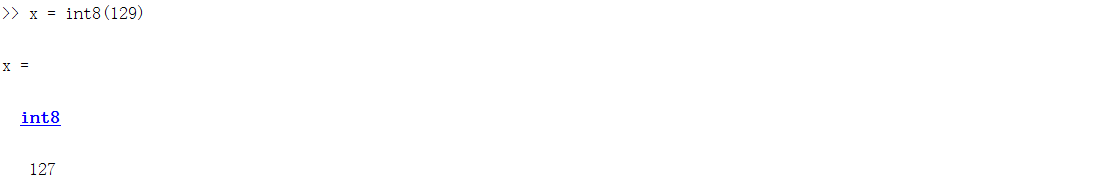int8函数将数值数据转换为带符号8位整数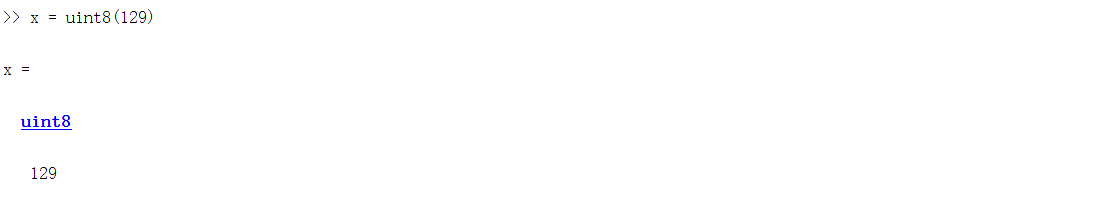1.2 浮点型
single函数：将其他类型数据转换为单精度型
double函数：将其他类型数据转换为双精度型
class函数：查看数据类型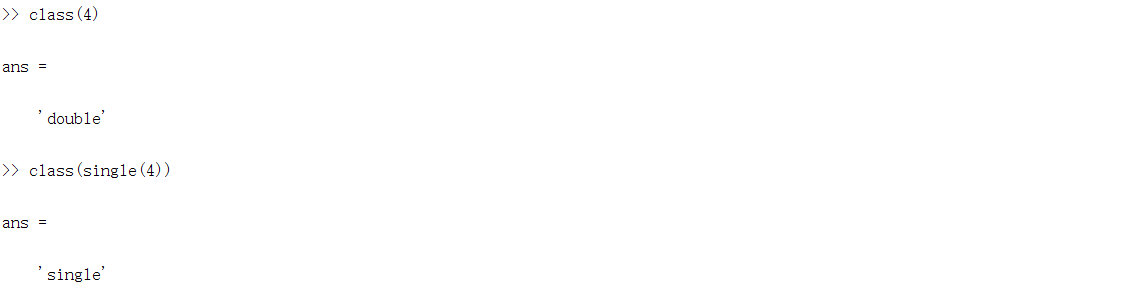1.3 复数型
复数分为实部和虚部，MATLAB中可用a+bi或者a+bj表示
real函数和imag函数分别表示查看数值的实部和虚部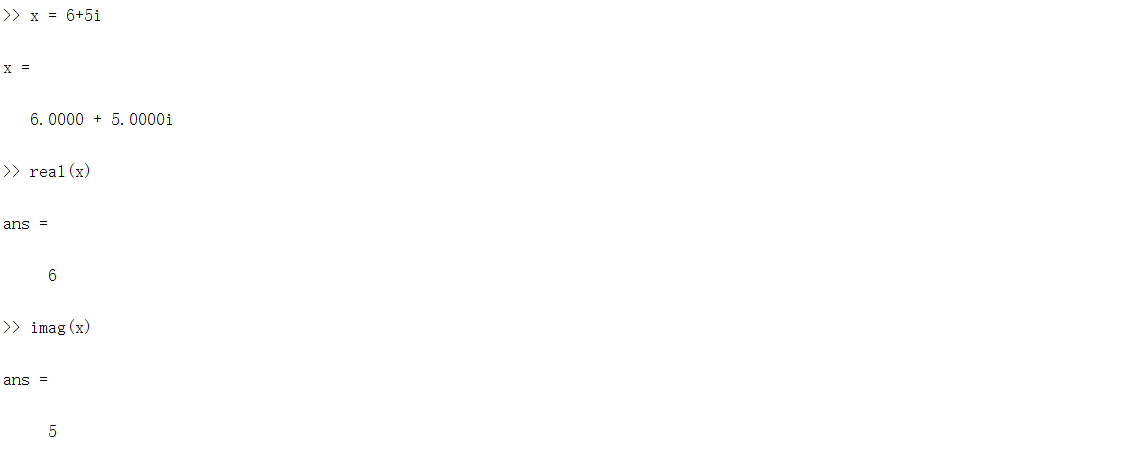1.4 数值数据的输出格式
format命令设置数值数据的输出格式
format命令后不加数据格式表示默认格式为short类型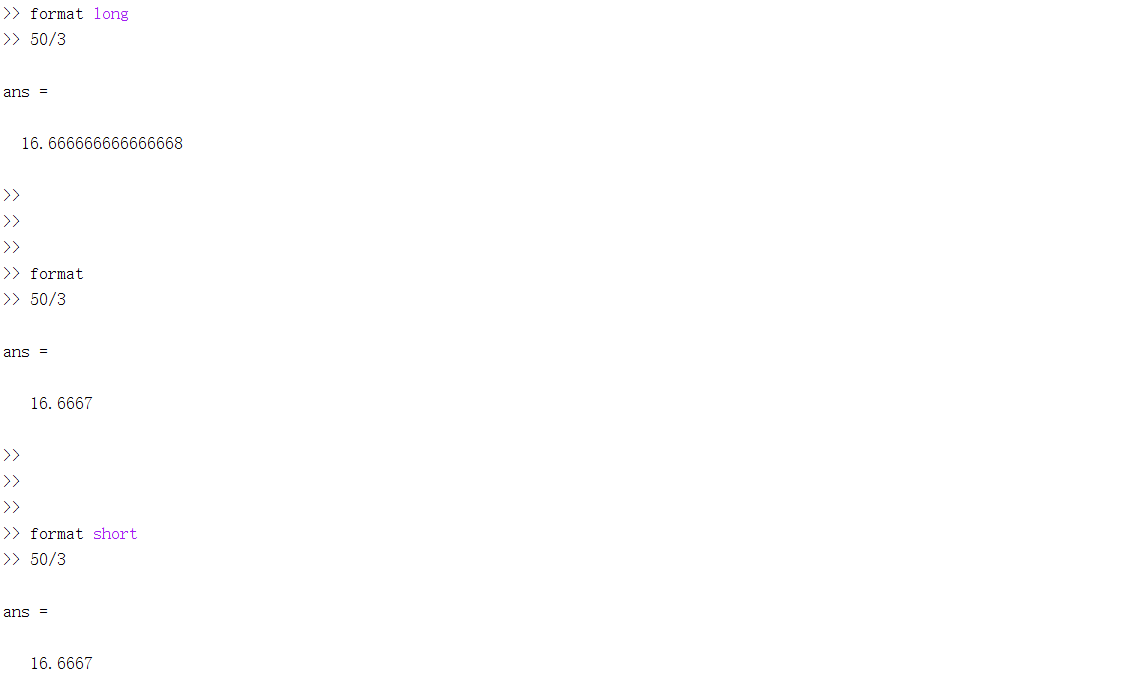1.5 常用数学函数
MATLAB中变量即为矩阵变量
定义变量时，逗号（，）用于分隔不同列，分号（；）用于分隔不同行。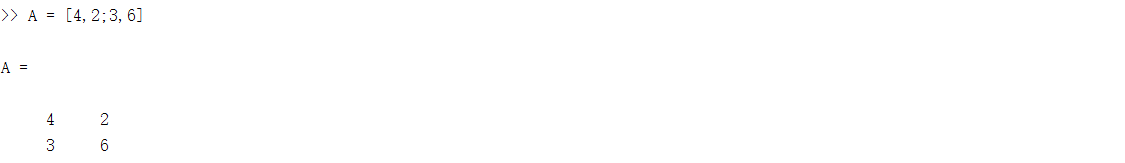常用函数如下表

exp(n)
e的n次方

sin (n)
n为弧度

sind(n)
n为角度

abs()
求实数的绝对值  复数的模  字符串的ASCII码值

round()
按四舍五入规则取整

ceil()
向上取整

floor()
向下取整

fix()
靠0取整

rem(m,n)
取余函数  取m/n的余数

isprime(n)
判断素数函数  n为素数时返回1否则0


展开全文• ## MATLAB学习笔记

千次阅读 多人点赞 2017-05-19 21:15:06
% Creation : 16-May-2017 9:50 % Last Revision : 15-Apr-2018 10:47 % Author : Lingyong Li {smilelingyong@163.com} % File Type : matlab % % Learning basic acknowledge of mat...
% Creation         : 16-May-2017 9:50
% Last Revision    : 15-Apr-2018 10:47
% Author           : Lingyong Li {smilelingyong@163.com}
% File Type        : matlab
%
% Learning basic acknowledge of matlab, and make some notes.
% -------------------------------------------------------------------------
% Lingyong Li @ 2017

%%  常见通用命令  P28
% clc                     清除命令窗口的显示内容
% clear                  清除Matlab工作空间中保存的变量
% clear + 变量名    清理内存指定变量
% clf                      清除图形窗口  clear figure
% who                   显示当前变量名列表
% whos                  显示变量详细列表
% which+函数名     证实该函数是否在当前路径
% what                  列出当前路径的所有matlab文件
% save                  保存文件到外部
% dir                     显示当前工作目录的文件和子目录清单
% cd                     显示或设置当前工作目录
% type                  显示指定m文件的内容
% help 或 doc        获取在线帮助
% lookfor+关键字   这个是matlab中的谷歌，模糊查询
% diary on
% diary off             记录命令行命令+运行结果，并以纯文本方式存储，方便编辑，正式版推荐使用publish
% %#ok                 除去编辑器的警告！！！
% quit 或 exit         关闭/推出MATALB

%%  必须牢记注意事项
% 1. 输入时，标点必须是英文状态下的
% 2. 大多数情况下，matlab对空格不予处理
% 3. 小括号代表运算级别，中括号用于生成矩阵，大括号用于构成单元数组
% 4. 分号;的作用：不显示运算结果，但对图形窗口不起作用。分号也用于区分行
% 5. 逗号,的作用：函数参数分隔符，也用于区分行，显示运算结果，当然不加标点也显示运算结果
% 6. 冒号:多用于数组
% 7. 续行号...不能放在等号后面使用，不能放在变量名中间使用，起作用时默认显蓝色
% 8. 双引号'string'是字符串的标识符
% 9. 感叹号!用于调用操作系统运算
% 10.百分号%是注释号，百分号后面直到行末的语句matlab跳过执行.另外还有一个块注释，即对多行一次注释，会使用到，格式为（注意%{ 和%}都要单独成行）
% %{
% %}
% 11.乘号*总是不能省略的，除了表示复数，比如2+3i时可以省略
% 12.除号/或\，它两个的关系是：a除以b表示为a/b，或b\a
% 13.等号=用于赋值
% 14.双等号==表示数学意义上的等号
% 15.主窗口里面，输入时，换行用Shift+Enter
% 16.主窗口里面，运行程序，执行命令用Enter
% 17.矩阵中用圆括号表示下标，单元数组用大括号表示下标
% 18.对变量名的基本要求：区分大小写，不超过63个字符，以字母开头，只能是字母，数字和下划线

% 19.clc即clear command（清屏），
% clf即clear figure（清理图形窗口）
% clear 清理内存所有变量，
% clear+变量名 清理内存指定变量
% edit+函数名 查看或编辑源文件
% who 显示当前变量名列表
% whos 显示变量详细列表
% which+函数名 证实该函数是否在当前路径
% what 列出当前路径的所有matlab文件
% save 保存文件到外部

% 20.matlab的帮助函数：
% help
% help+函数名或help+函数类名 精确查询
% helpwin 打开帮助窗口
% helpwin+函数名 精确查询
% helpdesk 打开帮助窗口
% doc 打开帮助窗口
% doc+函数名 打开帮助窗口， 精确查询
% lookfor+关键字 这个是matlab中的谷歌，模糊查询

% 21.有时候程序会陷入死循环，这时把操作切换到运行窗口，按Ctrl+C结束运行

% 22.函数式M文件的文件名，在matlab主窗口下不区分大小写，
% 函数式M文件中，变量都是局部变量
% 脚本式M文件中，变量都是全局变量

% 23.主窗口中，几个有用的快捷键：
% 在命令提示符后，可以用键盘上的上箭头和下箭头调用历史命令行
% Esc 清除当前输入行
% Ctrl+左箭头，光标左移一个单词
% Ctrl+右箭头，光标右移一个单词
% Alt+Backspace 恢复上次删除

% 24.编辑器（Editor）中的几个有用的快捷键：
% Tab或Ctrl+] 增加缩进，对多行有效
% Ctrl+[ 减少缩进，对多行有效
% Ctrl+I 自动缩进，对多行有效
% Ctrl+R 注释，对多行有效
% Ctrl+T 去掉注释，对多行有效
% Ctrl+B 括号配对检测，未配对会有红色波浪线标出
% F12 设置或取消断点
% F5 运行程序

%%   数据及其运算  P36
%% Matlab 预定义的变量
% ans      最近的计算结果的变量名
% pi         圆周率π
% e          exp
% eps      MATLAB定义的正的极小值=2.2204e-16
% inf        ∞值，无限大
% NaN     非数，0/0、∞/ ∞
% i或j       虚数单元，sqrt(-1)
%
% 注意：每当MATLAB启动完成，这些变量就被产生；
%       MATLAB中，被0除不会引起程序中断，给出报警的同时用inf或NaN给出结果。
%       用户只能临时覆盖这些预定义变量的值，Clear或重启MATLAB可恢复其值。

%%   数组相关知识  P45
%% 数组的创建
% 创建一维数组变量（行向量）三种方法：
% 1.使用方括号“[ ]”操作符
a=[1 3 pi 3+5i];  %or a=[1, 3, pi, 3+5i] ,创建数组(行向量)a=[1 3 pi 3+5i]
% 输出：a= 1.0000      3.0000      3.1416       3.0000 + 5.0000i
% 2.使用冒号“：”操作符（也等同于创建行向量）
b=1:10;  % 创建以1~10顺序排列整数为元素的行向量b.！！！注意：这里加（）或者加 [] 都可以得到同样的结果
% 输出：b=1 2 3 4 5 6 7 8 9 10
c=1:2:10;
% 输出：c=1 3 5 7 9
% 3.利用函数 linespace
% 函数linspace的基本语法
% x= linspace(x1, x2, n);
% 该函数生成一个由n个元素组成的行向量；
% x1为其第一个元素；
% x2为其最后一个元素；
% x1、x2之间元素的间隔=(x2-x1)/(n-1)。
% 如果忽略参数n，则系统默认生成100个元素的行向量。
x= linspace(1,2,5);     % 注意！！如果是直接在命令行输入，可以不用打“;”让其直接输出
% 输出：x=1.0000    1.2500    1.5000    1.7500    2.0000
% 4.利用函数 logspace (不常用)

% 创建列向量的方法：
% 1.使用方括号“[ ]”操作符，使用分号“;”分割行。
x= [1; 2; 3]
% 输出：X=1
%               2
%               3
% 2.使用冒号“：”操作符
x= (1:3)'    % “’”表示矩阵的转置，结果同上
x= [1:3]'

% 创建二维数组变量  P51
% 1.使用方括号“[ ]”操作符
% 使用规则
% 数组元素必须在“[ ]”内键入；
% 行与行之间须用分号“;” 间隔，也可以在分行处用回车键间隔；
% 行内元素用空格“ ”或逗号“,”间隔。
a2=[1 2 3;4 5 6;7 8 9];
a2=[1,2,3;4,5,6;7,8,9];
a2=[1:3;4:6;7:9];
% 结果：a2=
% 1  2  3
% 4  5  6
% 7  8  9
a=[1 2 3]; b=[2 3 4];  % 由向量构成二维数组
c=[a;b]
c=[a b]
% 2.函数创建方法
% 函数ones(生成全1矩阵)、zeros (生成全0矩阵) 、reshape
% “help elmat”获得基本的矩阵生成和操作函数列表
ones(3)     % 创建全1的 3*3数组
ones(3,4)   % 创建全1的 3*4数组
% reshape的使用方法
a=-4:4     % a= -4 -3 -2 -1 0 1 2 3 4
b=reshape(a,3,3)    % b=
%    -4  -1  2
%    -3   0  3
%    -2   1  4
% ！注意：数组元素的排列顺序，从上到下按列排列，先排第一列，然后第二列，… 要求数组的元素总数不变。

%% 数组元素的标识与寻访  P54 其中()圆括号用于访问数组元素
% 1.“全下标（index）”标识
% 二维数组
a(2,3); % 表示二维数组a的“第2行第3列”的元素
a(:,3); % 表示引用所有行的第3个元素，即引用第三列
%#%！注意“：”表示所有行或列，end表示最后一行或列------------------------

% 一维数组
b(2);   % 表示一维数组b的第二个元素

% 2.“单下标”（linear index）标识
% 即用一个下标来表明元素在数组中的位置
% ！！！注意：对于二维数组，将每一列连起来（列）！！！,然后从一开始数，其对应编号为其下标引用
a=zeros(2, 5);
a(:) = -4:5     % ！！！没看太懂(对每个数组元素赋值)
%     a =
%     -4    -2     0     2     4
%     -3    -1     1     3     5
% ！！注意:数组的排列顺序是按列先排

% 一维数组元素与子数组的寻访与赋值
a=linspace(1,10,5)
% a =
%     1.0000    3.2500    5.5000    7.7500   10.0000
a(3)   %寻访a的第3个元素
% ans =
%     5.5000
a([1 2 5])   %寻访a的第1、2、5个元素组成的子数组
% ans =
%     1.0000    3.2500   10.0000
a(1:3)   %寻访前3个元素组成的子数组
% ans =
%     1.0000    3.2500    5.5000
a(3:-1:1)  %由前3个元素 倒序 构成的子数组
% ans =
%     5.5000    3.2500    1.0000
a(3:end)
% ans =
%     5.5000    7.7500   10.0000
a(3:end-1)
% ans =
%     5.5000    7.7500
a([1 2 3 5 5 3 2 1])
% ans =
%     1.0000    3.2500    5.5000   10.0000   10.0000    5.5000    3.2500    1.0000
% 注意：数组元素可以被任意重复访问，构成长度大于原数组的新数组。
a(3)=0     % 修改数组a的第3元素值为0
% a =
%     1.0000    3.2500    0    7.7500   10.0000
% 注意：可以修改指定数组元素的值，一次可以修改多个数组元素的值，要修改的数组元素的个数应与送入数组的元素个数相同

% 二维数组元素与子数组的寻访与赋值
a_2=zeros(2, 4)  % 创建2x4的全0数组
% a_2 =
%      0     0     0     0
%      0     0     0     0
a_2(:)=1:8
% a_2 =
%      1     3     5     7
%      2     4     6     8      注意：按列排列
a_2([2 5 8])  %单下标方式寻访多个元素
% ans =
%      2     5     8
a_2([2 5 8]) = [10 20 30]  %单下标方式修改多个元素
% a_2 =
%      1     3    20     7
%     10     4     6    30
a_2(:,[2 3])=ones(2)  %双下标方式寻访并修改
% a_2 =
%      1      1     1    7
%      10     1     1    30
a_2(:,end)  %表示引用最后一列
% ans =
%      7
%     30
a_2(:,end-1) %表示引用倒数第二列
% ans =
%      1
%      1
a_2(:, end:-1:3) %表示引用从最后一列到第3列（倒序）
% ans =
%      7     1
%     30     1
a_2(end,:)      %表示引用最后一行
% ans =
%     10     1     1    30
a_2(end,[2:4])  %表示引用最后一行中第 2-4个元素
% ans =
%      1     1    30
a_2([4 6])=6:7  %表示将二维数组单下标赋值，对第4-6个元素赋值
% a_2 =
%      1     1     1     7
%     10     6     7    30
a_2(end,[2:end-1]) %表示引用最后一行，第2-倒数第二个元素
% ans =
%      6     7
%注意：二维数组可以“单下标”方式或“全下标”方式访问、赋值；
%“单下标”方式赋值时，等号两边涉及的元素个数必须相等；
%“全下标”方式赋值时，等号右边数组的大小必须等于原数组中涉及元素构成的子数组的大小。

% size、length函数
a=ones(4,6)*6   %创建一个4*6的数组，且每个元素均为6
m=size(a)   %size函数返回变量的大小，即变量数组的行列数
% m =
%      4     6
b = 1:5     %创建一个一维数组
length(b)   %length函数返回变量数组的最大维数 为5
c = b'
length(c)   %维数也为5

% 双下标转换为单下标，sub2ind函数  % 重要!!!！
A = [17 24 1 8; 2 22 7 14; 4 6 13 20];%创建一个二维数组
% 这里使用了三维数组的寻址方式
A(:,:,2) = A - 10  %！！将二维数组中的每个元素 -10,用这种方式是为了看到最初的A数组，以及修改后的A，最直接的办法就是 A = A - 10，后面就可以直接引用A(2,1)访问 -8这个元素
% A(:,:,1) =
%     17    24     1     8
%      2    22     7    14
%      4     6    13    20
% A(:,:,2) =
%      7    14    -9    -2
%     -8    12    -3     4
%     -6    -4     3    10
A(2,1,2)   %访问第二个数组，中的第二行，第一个元素，-8
% ans = -8
sub2ind(size(A),2,1,2)  %使用sub2ind函数将双下标转换为单下标
% ans = 14
A(14)
% ans = -8  其结果与A(2,1,2)一致

% 单下标转换为双下标，ind2sub函数
b = zeros(3) %创建一个3*3元素为0的数组变量b
b(:)=1:9     %对数组元素赋值
% b =
%      1     4     7
%      2     5     8
%      3     6     9
index = 4   %设置index为要转换的单下标4，其对应元素正好也为4
[I,J]=ind2sub(size(b),index)
% I =       对应其双下标方式的列下标
%      1
% J =       对应其单下标方式的列下标
%      2
IND = [3 4 5 6] %也可以同时转换多个单下标为双下标
% I =
%      3     1     2     3
% J =
%      1     2     2     2

%% 多维数组  P66
% 在 MATLAB的数据类型中，向量可视为一维数组，矩阵可视为二维数组，对于维数(Dimensions)超过2 的数组均可视为「多维数组」
% 将两个二维（平面）数组叠在一起，就构成三维数组，第三维称为「页」(Page)
% 以维数为 3×4×2 的三维数组为例:
% A(：,：,1)代表第一页的二维数组
% A(：,：,2)代表第二页的二维数组

A(:, :, 1) = [1 0 2 5; 4 1 8 7; 3 2 6 3];
A(:, :, 2) = [3 5 4 1; 2 6 2 1; 4 2 3 0]  % 由两个相同大小二维数组创建三维数组
% A(:,:,1) =
%      1     0     2     5
%      4     1     8     7
%      3     2     6     3
%
% A(:,:,2) =
%      3     5     4     1
%      2     6     2     1
%      4     2     3     0
whos A    %得到变量A的详细信息
%   Name      Size             Bytes  Class     Attributes
%   A         3x4x2              192  double

%%  数组的算术运算  P72
% MATLAB数组运算符列表
% 运算                运算符		 含义说明
% 加                      +          相应元素相加
% 减                       -		     相应元素相减
% 乘                       *		     矩阵乘法
% 点乘                   .* 	       相应元素相乘
% 幂                       ^ 		  矩阵幂运算
% 点幂	  	 	       .^		   相应元素进行幂运算
% 左除或右除		    \或/	       矩阵左除或右除
% 左点除或右点除  .\或./	  A的元素被B的对应元素除

% 加法 “+” ===========================
a = zeros(2,3)
a(:)=1:6;
b=a+2.5
%  b =
%       3.5000    5.5000    7.5000
%       4.5000    6.5000    8.5000
c=b-a
% c =
%       2.5000    2.5000    2.5000
%       2.5000    2.5000    2.5000

% 画出y=1/(x+1)的函数曲线，x∈[0, 100]
x=0:100;
y=1./(x+1);
plot(x, y);
legend( 'y=1/(x+1)');   % 添加图例，为图像添加说明

% 生成一个信号：x=sin(2*pi*t)+sin(4*pi*t)
t = [0:199]./100;      %采样时间点
% 生成信号
x = sin(2*pi*t) + sin(4*pi*t);
plot(t,x);
legend( 'x = sin(2*pi*t) + sin(4*pi*t)' );

% 点幂 “.^” （即每个元素的幂次）===========
a=1:6
% a =
%      1   2   3   4   5  6
b=reshape(a,2,3)
% b =
%      1     3     5
%      2     4     6
a=a.^2
% a =
%      1   4   9   16   25  36
b=b.^2
% b =
%      1     9     25
%      4     16    36

%% 关系运算   P79
%  Matlab提供了6种关系运算符： <、>、<=、>=、==、~=（不等于）
% 关系运算符的运算法则：
%       1、当两个标量进行比较时，直接比较两数大小。若关系成立，果为1，否则为0。
%       2、当两个维数相等的矩阵进行比较时，其相应位置的元素按标量关系进行比较，并给出结果，形成一个维数与原来相同的0、1矩阵。
%       3、当一个标量与一个矩阵比较时，该标量与矩阵的各元素进行比较，结果形成一个与矩阵维数相等的0、1矩阵。

% 建立5阶方阵A，判断其元素能否被3整除
A = [24, 35, 13, 22, 63; 23, 39, 47, 80, 80; ...
90, 41, 80, 29, 10; 45, 57, 85, 62, 21; 37, 19, 31, 88, 76]
% A =
%       24    35    13    22    63
%       23    39    47    80    80
%       90    41    80    29    10
%       45    57    85    62    21
%       37    19    31    88    76
P = rem(A,3)==0     %被3除，求余
% P =
%      1     0     0     0     1
%      0     1     0     0     0
%      1     0     0     0     0
%      1     1     0     0     1
%      0     0     0     0     0

%% 逻辑运算   P81
%    Matlab提供了3种逻辑运算符：&（与）、|（或）、~（非）
% 逻辑运算符的运算法则：
%        1、在逻辑运算中，确认非零元素为真（1），零元素为假（0）。
%        2、当两个维数相等的矩阵进行比较时，其相应位置的元素按标量关系进行比较，并给出结果，形成一个维数与原来相同的0、1矩阵；
%        3、当一个标量与一个矩阵比较时，该标量与矩阵的各元素进行比较，结果形成一个与矩阵维数相等的0、1矩阵；
%        4、算术运算优先级最高，逻辑运算优先级最低。

% 在[0，3 π]区间，求y = sin x 的值。要求消去负半波，即(π,2π)区间内的函数值置零。
x = 0:pi/100:3*pi;
y = sin(x);
y1 = (y>=0).*y;   %消去负半波
plot(x,y1)            %画好图

% 建立矩阵A，找出在[10，20]区间的元素的位置。
A = [4,15,-45,10,6;56,0,17,-45,0];
find(A>=10 & A<=20)     %找到满足要求的元素位置 !!!注意这里要使用 & 而不是 &&（但自己未去百度！！（补充））

%% 最大值和最小值   P84
%  1、求向量的最大值和最小值
%        求一个向量X的最大值的函数有两种调用格式，分别是：
%       (1)  y=max(X)：返回向量X的最大值存入y，如果X中包含复数元素，则按模取最大值；
%       (2)  [y,I]=max(X)：返回向量X的最大值存入y，最大值的序号存入I，如果X中包含复数元素，则按模取最大值。
%       求向量X的最小值的函数是min(X)，用法和max(X)完全相同。
% 【例7-1】求向量的最大值
x=[-43,72,9,16,23,47];
y=max(x)     %求向量x中的最大值
% y =
%      72
[y,l]=max(x)    %求向量x中的最大值及其该元素的位置
% y =
%     72
% l =
%     2

%  2、求矩阵的最大值和最小值  （！多看几遍）
%       求矩阵A的最大值的函数有3种调用格式，分别是：
%       (1) max(A)：返回一个行向量，向量的第i个元素是矩阵A的第i列上的最大值；
%       (2) [Y,U]=max(A)：返回行向量Y和U，Y向量记录A的每列的最大值，U向量记录每列最大值的行号；
%       (3) max(A,[],dim)：dim取1或2。dim取1时，该函数和max(A)完全相同；dim取2时，该函数返回一个列向量，其第i个元素是A矩阵的第i行上的最大值。
%       求最小值的函数是min，其用法和max完全相同。

%  【例7-2】求矩阵的最大值
x=[-43,72,9; 16,23,47];
y=max(x)     %求矩阵x中每列的最大值
% y =
%      16  72  47
[y,l]=max(x) %求矩阵x中每列的最大值及其该元素的位置
% y =
%     16  72  47
% l =
%      2  1  2
max(x, [],1)   %求矩阵中每列的最大值
max(x, [],2)   %求矩阵中每行的最大值

%% 求和与求积  （未细看） P88
% sum(X)：返回向量X各元素的和。
% prod(X)：返回向量X各元素的乘积。
% sum(A)：返回一个行向量，其第i个元素是A的第i列的元素和。
% prod(A)：返回一个行向量，其第i个元素是A的第i列的元素乘积。
% sum(A,dim)：当dim为1时，该函数等同于sum(A)；当dim为2时，返回一个列向量，其第i个元素是A的第i行的各元素之和。
% prod(A,dim)：当dim为1时，该函数等同于prod(A)；当dim为2时，返回一个列向量，其第i个元素是A的第i行的各元素乘积。

%% 平均值mean 与 中值 median （未细看） P89
% mean(X)：返回向量X的算术平均值。
% median(X)：返回向量X的中值。
% mean(A)：返回一个行向量，其第i个元素是A的第i列的算术平均值。
% median(A)：返回一个行向量，其第i个元素是A的第i列的中值。
% mean(A,dim)：当dim为1时，该函数等同于mean(A)；当dim为2时，返回一个列向量，其第i个元素是A的第i行的算术平均值。
% median(A,dim)：当dim为1时，该函数等同于median(A)；当dim为2时，返回一个列向量，其第i个元素是A的第i行的中值。

%%     字符串  P90
% 关于字符串
%       MATLAB处理字符(Characters)与字符串(Strings)的相关指令大部分都放在下列目录之中：{MATLAB根目录}\toolbox\matlab\strfun
% 其中的「strfun」就是代表「String Functions」。若要查询与字符和字符串相关的指令，可在 MATLAB 下输入：help strfun 或是 help strings
% 字符（Characters）可以构成一个字符串（Strings），或字符数组(character array)。 一个字符串是被视为一个行向量（row vector）。
% 字符串中的每一个字符（含空格），以其 ASCII 码的形式存放于行向量中，是该字符串变量的一个元素（element）。
% Matlab 用「单引号」来界定一个字符串。

% 可以使用方括号“[ ]”直接连接多个字符串变量，得到一个新字符串变量。
% 【例1】命名字符串变量
str1 = ' I like MATLAB,';	    % 建立字串变量 str1
str2 = ' JavaScript, and Perl!';	    % 建立字串变量str2
str3 = [str1 str2]	        % 直接连接str1及str2，以建立str3
% str3 =
%      I like MATLAB, JavaScript, and Perl!

% 如要输入的字符串中有单引号，则由两个连续的单引号来表示。
% 若要计算字符串变量的长度（即组成字符串的个数），可用 length 指令。
% 【例2】含单引号字符串的输入
sentence = 'I''ve got a date!';
length(sentence)	% 计算字字符串sentence的长度

% double 指令: 查看字符串变量的存储內容（即 ASCII 内码）
% char     指令: 将 ASCII 內码转换为字符串形式
% 【例3】 : 字符串与ASCII码的相互转换 （不熟）！
sentence = 'I''ve got a date!';
sentenceAscii = double(sentence)   %查看 sentence 的 ASCII 码
sentence2 = char(sentenceAscii)	     % 将 ASCII 码恢复成字符串形式

% 字符串的判断
% class 或 ischar 指令: 可以用来判断一个变量的类型或它是否为字符串变量。
% 【例4】 : 判断一个变量是否为字符串变量
chinese = '今日事，今日毕';
out1 = class(chinese)      % out1 的值是 “char”
x = chinese+1;
out2 = ischar(x)	           % out2 的值是 0，代表 x 不是字符串变量

% 一个字符数组变量存储多行字符串 （多看几遍）！
% 1、使用二维字符数组（Two Dimensional Character Arrays）
% 必须先确认每个字符串（即每一行）的长度一样，否则就必须在短字符串结尾补齐空格
% 2、使用char指令存储多字符串
% 【例5】 : 多行字符串变量
departments = ['aa ';'bb ';'ccc']        %使用分号隔开，并且每一行的长度要一致才行,这里aa bb 后面都补了一个空格，保证每行长度一致
departments = char('aa','bb','ccc')    %使用逗号隔开，结果与上面一致
%     departments =
%     aa
%     bb
%     cc
% ！注意：从二维字符数组访问字符串时，切记要使用 deblank 指令来清除字符串尾部的空格字符
% 【例6】 : 使用deblank命令清除字符串尾部空格 （未看太懂）
departments = char('aa', 'bb', 'ccc');
dept1 = departments(1,:);	% (1,:)代表第一行的全部元素  "aa "
dept2 = deblank(dept1);	     % 使用 deblank 指令清除尾部的空格字符
len1 = length(dept1)		    % 显示变量 dept1 的长度=3
len2 = length(dept2)		    % 显示变量 dept2 的长度=2

% strcmp 指令: 用于比较字符串的內容的异同 .字符串比不相等返回0,相等返回1
str1 = 'today';
str2 = 'tomorrow';
str3 = 'today';
out1 = strcmp(str1, str2)	   % 比较字符串 str1 和 str2
%  out1 = 0                         %表示字符串 str1 和 str2不同
out2 = strcmp(str1, str3)	   % 比较字符串 str1 和 str3
%  out2 = 1                         %表示字符串 str1 和 str2相同

%%      数组补充知识
%%  空数组     P99
% 有一维是0的数组即为空数组
% 空数组不占据存储空间
% 最简单的空数组：0 x 0的矩阵
% 复杂的空数组：0 x 5 or 10 x 0
a=[]; b=ones(0,5);
% 察看空数组：
a, b, c    % or  whos a b c
% 空数组并非全0数组

%%   数组维数的减小 （多看几遍）P100
% 删除数组的某列和行
a = magic(4), a(:,2)=[] %使用magic函数创建一个4*4的数组，然后删除第二列,自己doc magic函数用法
% 删除(2-D、3-D)数组的单个元素
%使用“全下标”方式，不能删除单个元素（注意！）
a(1, 2)=[]   %系统会警告信息
%使用“单下标”可以删除单个元素 （注意！）
a(2:4)=[]   %这里删除了下标从2—4的元素，然后将数组a变为向量
% 使用“[]”同样可以减小字符数组的维数

%%    元胞数组（单元数组） P101
%% 基本概念
% 特殊的数据类型，在一个数组中存放各种不同类型的数据
% 每个单元相当于一个“盒子”
% “盒子”可存储各种不同类型的MATLAB数据
% 元胞数组例子
%  Cell 1,1        Cell 1,2        Cell 1,3
%  Taiwan        Mainland       [1 2 3 4]
%  Taibei          Beijing             test
%% 创建方法
%      1、赋值语句
%      2、cell函数

% 1.赋值语句创建元胞数组
%   （1.元胞索引(cell indexing)方式
%         格式：a(1, 2)={… … …}
%   （2.元胞内容索引(content indexing)方式
%        格式：a{1, 2}=[…]  or ‘…’
%   （3.直接用大括号一次把所有元素括起来
b = {'James Bond', [1 2;3 4;5 6]; pi, ones(5)}

% 2. Cell indexing方式创建元胞数组
a(1,1) = {[1 4 3; 0 5 8; 7 2 9]};
a(1,2) = {'Anne Smith'};
a(2,1) = {3+7i};
a(2,2) = {-pi:pi/10:pi};    %通过查看数组中的值一下就可以明白
% 其中“{ }” 表示空元胞数组
% 3.Content indexing方式创建元胞数组
b{1,1} = 'James Bond' ;
b{1,2} = [1 2;3 4;5 6];
b{2,1} = pi;
b{2,2} = zeros(5);

% 4. cell函数创建元胞数组 （注意：这里是自己为了方便看，将其从PPT的后部分插到这前面的部分了！）
d = cell(2, 3)
%     d =
%          []     []     []
%          []     []     []
whos d
%     Name      Size            Bytes  Class    Attributes
%     d              2x3                48     cell
% 注意：每个cell占有4个字节的空间
d(1,3) = {1:3};  % or d{1,3} = 1:3;    将数组第1行第3列的数组元素进行初始化

%% 显示元胞数组的内容
% celldisp显示元胞数组的全部内容
a
% a =
%     [3x3 double]             'Anne Smith'
%     [3.0000 + 7.0000i]    [1x21 double]
celldisp(a)  %显示全部内容
cellplot(a)   %图形方式显示元胞数组的结构，自己动手练一遍！

% 使用内容下标索引显示指定元胞的数据
% 格式：var_name{row_index, column_index}
%          或
%          var_name{:}
% Example
a{1, 2}
%     ans =
%     Anne Smith
a{:}          %一次显示a的全部数据
% ans =
%     [3x3  double]
%     [3.0000 + 7.0000i]
%     'Anne Smith'
%     [1x21 double]

% 读取元胞数组中的内容
%    直接取用元胞数组的整个元胞（单元）
%    取用元胞数组某元胞內的数据单位
%    一次读取或刪除多个元胞
%【例1】读取上述b元胞数组的第1行、第2列元胞的内容
b,e=b{1,2}   %注意！逗号的作用是先不将b的结果显示出来
%     b =
%         'James Bond'    [3x2 double]    [1x3 double]
%         [    3.1416]    [5x5 double]              []
%     e =
%
%          1     2
%          3     4
%【例2】读取b{1, 2}的第3行、第1列的数据
f = b{1,2}(3,1)
%     f =
%          5
%#%  注意！读取元胞数组元胞内部分数据：
%#%            元胞数组内容索引，再加上一般数组的索引，
%#%            即：var_name {row_ind,col_ind} (r_ind, c_ind) ，即先大括号再小括号

%【例3】读取元胞数组a第1行的所有元胞
f=a(1,:)
%     f =
%          [3x3 double]    'Anne Smith'
%【例4】删除元胞数组a第1行的所有元胞
a(1,:) = []
%     a =
%     [3.0000 + 7.0000i]    [1x21 double]
%#%  注意！ Note: 这里使用的是元胞索引（cell indexing），而不是内容索引（content indexing）

%% 元胞数组的连接
% 【例】连接元胞数组a、b，生成元胞数组c
clear c
c=[a b]     % 列连接
%     c =
%     [3x3 double]          'Anne Smith'     'James Bond'    [3x2 double]
%     [3.0000 + 7.0000i]  [1x21 double]    [    3.1416]    [5x5 double]
c=[a; b]     % 行连接
%     c =
%     [3x3 double]               'Anne Smith'
%     [3.0000 + 7.0000i]       [1x21 double]
%     'James Bond'              [3x2  double]
%     [          3.1416]           [5x5  double]

%%     结构与结构数组
%% 基本概念
% 1.结构(structure)
%       由字段（或域，fields）组成
%       每个字段可以是任一种Matlab数据类型的数据或变量
%       与C语言的结构类型相似
% 2.结构数组(structure array)
%       多个结构构成结构数组(structure array)
%       结构数组的元素就是一个结构

% 结构数组变量的创建
% 方法一：直接键入
% 【例1】创建一个关于学生信息的结构数组，每个结构包含学生姓名(name)、学号(id)、成绩(scores)。
clear student			% 清除 student 变量
student.name = '张三';		% 加入 name 字段
student.id = 'mr871912';		% 加入 id字段
student.scores = [58, 75, 62];	% 加入 scores字段
student				% 显示结构变量的数据
%     student =
%            name: '张三'
%                 id: 'mr871912'
%          scores: [58,75,62]
% 此时，Matlab视 student 为一个1x1结构数组

% 第二个学生信息的建立
% 此时，student变量已成为一个1*2的结构数组
student(2).name = '张宁';	  % 加入 name 字段
student(2).id = 'mr871913';	  % 加入 id字段
student(2).scores=[68, 85, 92];       % 加入 scores字段
student				   % 只是简单输入变量名student的话，Matlab不在显示器所有的数据信息，只是给出结构
%   student =
%           1x2 struct array with fields:
%            name
%            id
%            scores
student(1)          % 显示第一个同学的信息
student(2)          % 显示第二个同学的信息

% 方法二：struc函数
% 格式：
%         Structure Array_var_name = struct(field1, value1, field2, value2,….)
%         field1、field2、…是结构的字段名，
%         value1、value2、…则是相应字段所包含的数据
clear student
student=struct('name', '张听说', 'scores', [50 60]);
student(2)=struct('name', '张廷安', 'scores', [60 70]);
student(1), student(2)   %显示student(1),student(2)
%      ans =
%           name: '张听说'
%         scores: [50 60]
%     ans =
%           name: '张廷安'
%         scores: [60 70]
%  或者使用struct创建结构数组变量（一次建立多个元素）
clear student
student = struct('name', {'张听说', '张廷安'}, 'scores', {[50 60], [60 70]});
student(1), student(2)   %显示student(1),student(2)

% 结构数组嵌套（nested）
% 结构数组中结构的字段还可以是结构
student = struct('name', {'张听说', '张廷安'}, 'scores',{[55 60], [65 80]});
student(2).course(1).title = 'Web Programming';
student(2).course(1).credits = 2;
student(2).course(2).title = 'Numerical Method';
student(2).course(2).credits = 3;
student(2).course
% 访问结构数组变量的内容 (通过使用 . 的方式访问)
student_name = student(1).name
% student_name =  张听说
% 修改结构数组变量的字段
student(1).name='Newton';

% cat函数：排列结构数组某字段的值
%       格式：cat(dim, structurefield)
%       dim=1, 竖排；dim=2, 横排
cat(1, student.scores)
%     ans =
%         55    60
%         65    80
cat(2, student.scores)
%     ans =
%         55    60    65    80

% mean函数计算平均值
% 计算每次考试的平均值
average1=mean(cat(1, student.scores))   %结果没看太懂，要回头看mean函数（注意！）
%      average1 =
%           60    70

%% 数据以及函数的可视化  （重点，难点！！！）
%% plot指令的基本调用格式  P128
% （1）plot(x)
%            x为向量时，以该元素的下标为横坐标、元素值为纵坐标绘出曲线
%            x为实数二维数组时，则按列绘制每列元素值相对其下标的曲线，曲线数等于x数组的列数。
%            x为复数二维数组时，则按列分别以数组的实部和虚部为横、纵坐标绘制多条曲线
% （2）plot(x, y)
%            x、y为同维数组时，绘制以x、y元素为横纵坐标的曲线
%            x为向量，y为二维数组、且其列数或行数等于x的元素数时，绘制多条不同颜色的曲线
%            x为二维数组，y为向量时，情况与上相同，只是y仍为纵坐标。
% （3）plot(x1, y1, x2, y2, …)
%           绘制以x1为横坐标、y1为纵坐标的曲线1，以x2为横坐标、y2为纵坐标的曲线2，等等。
%           其中x为横坐标，y为纵坐标，绘制y=f(x)函数曲线。

% 【例1】在[0，2π]区间内，绘制曲线 y = 2*exp(-0.5*x).*sin(2*pi*x);  P129
x = 0:pi/100:2*pi;
y = 2*exp(-0.5*x).*sin(2*pi*x);
plot(x,y)
% 【例2】画出如图所示：  P130
t = -pi:pi/100:pi;
x = t.*cos(3*t);
y = t.*sin(t).^2;
plot(x,y)
% 【例3】用图形表示连续调制波形 y=sin(t)sin(9t) 及其包络线（没看太懂！细看多看几遍）
t=(0:pi/100:pi)' ;		%长度为101的时间采样列向量
y1=sin(t)*[1,-1];		%包络线函数值，是（101x2）的矩阵
y2=sin(t).*sin(9*t);	%长度为101的调制波列向量
t3=pi*(0:9)/9;
y3=sin(t3).*sin(9*t3);
plot(t,y1,'r:',t,y2,'b',t3,y3,'bo')
axis([0,pi,-1,1])		%控制轴的范围

% 多次叠绘      P132
% 多次调用plot命令在一幅图上绘制多条曲线，需要hold指令的配合。
hold on   保持当前坐标轴和图形，并可以接受下一次绘制。
hold off   取消当前坐标轴和图形保持，这种状态下，调用plot绘制完全新的图形，不保留以前的坐标格式、曲线。
% 采用叠加绘制的方法实现上面的图形
t=(0:pi/100:pi)';	 %长度为101的时间采样列向量
y1=sin(t)*[1,-1];	%包络线函数值，是（101x2）的矩阵
y2=sin(t).*sin(9*t);	%长度为101的调制波列向量
t3=pi*(0:9)/9;
y3=sin(t3).*sin(9*t3);
plot(t,y1,'r:')
hold on
plot(t,y2,'b')
plot(t3,y3,'bo')
axis([0,pi,-1,1])		%控制坐标轴的范围
hold off
% 利用hold绘制离散信号的波形
t=2*pi*(0:20)/20;       %也要熟悉这种分割
y=cos(t).*exp(-0.4*t);
stem(t,y,'g');
hold on;
stairs(t,y,'r');
hold off

%% plotyy指令：双纵坐标   P135
% 格式：
%         plotyy(x1, y1, x2, y2)
%         x1-y1曲线y轴在左， x2-y2曲线y轴在右。
% 【例1】
x = 0:0.01:20;
y1 = 200*exp(-0.05*x).*sin(x);
y2 = 0.8*exp(-0.5*x).*sin(10*x);
plotyy(x,y1,x,y2);

%% subplot指令：多子图    P136  （不熟悉，多看几遍！）
% 在同一图形窗口布置几幅独立的子图
% subplot(m, n, k)
%        使（m * n）幅子图中第k个子图成为当前图
% subplot(‘postion’, [left, bottom, width, height])
%        在指定的位置上开辟子图，并成为当前图
% 说明：
%       subplot(m, n, k)的含义：图形窗口包含（mxn）个子图，k为要指定的当前子图的编号。其编号原则： 左上方为第1子图，然后向右向下依次排序。该指令按缺省值分割子图区域。
%       subplot(‘postion’, [left, bottom, width, height])用于手工指定子图位置，指定位置的四元组采用归一化的标称单位，即认为整个图形窗口绘图区域的高、宽的取值范围都是[0， 1]，而左下角为（0,0）坐标。
%       产生的子图彼此独立。所有的绘图指令均可以在子图中使用。
%【例1】
t=(pi*(0:1000)/1000)';
y1=sin(t);y2=sin(10*t);y12=sin(t).*sin(10*t);
subplot(2,2,1),plot(t,y1);axis([0,pi,-1,1])
subplot(2,2,2),plot(t,y2);axis([0,pi,-1,1])
subplot('position',[0.2,0.05,0.6,0.45])
plot(t,y12,'b-',t,[y1,-y1],'r:');
% 【例2】Specify Line properties （画出来的图蛮好看的，多画画）
t=(0:15)*2*pi/15;
y=sin(t);
subplot(3,2,1), plot(t, y); title('Lins style is default')
subplot(3,2,2), plot(t, y, 'o'); title('Lins style is o')
subplot(3,2,3), plot(t, y, 'k:'); title('Lins style is k:')
subplot(3,2,4), plot(t, y, 'k:*'); title('Lins style is k:*')
subplot(3,2,5), plot(t, y, 'm-d'); title('Lins style is m-d')
subplot(3,2,6), plot(t, y, 'r-p'); title('Lins style is r-p')
% 【例3】绘制椭圆，长轴为3.25，短轴为1.15
t=0:2*pi/99:2*pi;
x=1.15*cos(t); y=3.25*sin(t);    % y为长轴，x为短轴
subplot(2,2,1); plot(x, y);
axis off
title('axis off');
subplot(2,2,2); plot(x,y);
axis image;
title('axis image');
subplot(2,2,3); plot(x,y);
axis equal;
title('axis Equal');
subplot(2,2,4); plot(x,y);
axis square;
title('axis Square');

%% 画图常用标记   P138
% 曲线线形控制符
-   实线            %#ok
:   虚线            %#ok
-.  点划线          %#ok
--  双划线          %#ok

% 曲线颜色控制符
b   兰
g   绿
r   红
c   青
m  品红
y   黄
k   黑
w   白
%  数据点形控制符
.    实心黑点     %#ok
+   十字符        %#ok
*    八线符        %#ok
^    朝上三角     %#ok
<   朝左三角     %#ok
>   朝右三角     %#ok
v    朝下三角
d    菱形符（diamond）
h    六角星符（hexagram）
o    空心圆圈
p    五角星符（pentagram）
s    方块符（square）
x    叉字符
%注意：曲线的线形控制符、颜色控制符、数据点形控制符可以组合使用
%其先后次序不影响绘图结果；也可以单独使用。

%% 常用坐标控制指令   P142
axis auto           使用缺省设置
axis manual       使当前坐标范围不变
axis off               不显示坐标轴
axis on               显示坐标轴
axis ij                 坐标原点在左上方
axis xy               坐标原点在左下方
axis(v)
v=[x1,x2,y1,y2]       设定坐标范围          %#ok
axis([[x1,x2,y1,y2]) 等同于上面两句       %#ok  （最常用！！！）
axis equal          纵横坐标等长刻度
axis image         纵横坐标等长刻度，坐标框紧贴数据范围
axis square        产生方形坐标系
axis normal         缺省矩形坐标系
%% 刻度、分割线、坐标框  P145
%分割线与grid指令
grid on                 画出分割线
grid off                 不画分割线
%注意：MATLAB的缺省设置是不画分格线；分格线的疏密取决于坐标刻度（改变坐标刻度，可改变分格线的疏密）。
%坐标框
box on                  控制加边框线
box off                  控制不加边框线
%刻度设置
set(gca, 'xtick', xs, 'ytick', ys)
%其中：xs、ys可以使任何合法的实数向量，用于分别设置x、y轴的刻度。

%【例1】绘制y=1-exp(-0.3*t).*cos(0.7*t)
t=6*pi*(0:100)/100;
y=1-exp(-0.3*t).*cos(0.7*t);
tt=t(find(abs(y-1)>0.05));   % tt中保存所有满足条件的y
ts=max(tt);                      % ts中保存tt中y的最大值
plot(t,y,'r-');     %绘制曲线
grid on;          %绘制分割线
axis([0,6*pi,0.6,max(y)]);      %画坐标范围
title('y=1-exp(-\alpha*t)*cos(\omega*t)');
hold on;
plot(ts,0.95,'bo');
hold off;
set(gca,'xtick',[2*pi,4*pi,6*pi],'ytick',[0.95,1,1.05,max(y)]);      %（未看完） 设置网格的显示格式,gca获取当前figure的句柄
grid on;

%% 图形标识  P149
% 图形标识包括：
%       图名（title）
%       坐标轴名（xlabel、ylabel）
%       图形文本注释（text）
%       图例（legend）
% 简捷使用格式
%       title(s)		  % s为字符串变量或常量
%       xlabel(s)
%       ylabel(s)
%       legend(s)
%       text(x, y, s)	% 指定坐标（x, y）处加注文字

% 字体样式设置： P150 （很不熟，多看几遍！）
\fontname{arg} \arg \fontsize {arg} string
%其中，String为要输出的字符串，其前面的均为属性控制，使用方法见下表。

%【例1】
t=(0:100)/100*2*pi;
y=sin(t);
plot(t, y)
text(3*pi/4,sin(3*pi/4), '\fontsize{16}\leftarrowsin(t) = .707 ')
text(pi, sin(pi), '\fontsize{16}\leftarrowsin(t) = 0 ')
text(5*pi/4, sin(5*pi/4), '\fontsize{16}sin(t) = -.707\rightarrow',...  %注意...是换行连接
'HorizontalAlignment','right')    %其中‘HorizontalAlignment’, ‘right’设置图形标识为水平右对齐
%【例2】
t = 0:900;
plot(t,0.25*exp(-0.005*t))
title('\fontsize{16}\itAe^{\alphat}');
text(300,.25*exp(-0.005*300),...
'\fontsize{14}\leftarrow0.25\ite^-0.005\itt at \itt = 300');

%% 特殊图形   P154
%% 直方图(柱形图)bar
%       垂直直方图
%              累计式
%              分组式
%       水平直方图
%              累计式
%              分组式

% 【例1】柱状图
x = -2.9:0.2:2.9;
bar(x,exp(-x.*x),'r')

% 【例2】累积直方图+分组直方图+
%             北京市从业人员统计
%                     1990年	   1995年	 2000年
% 第一产业 	       90.7		  70.6		  73.9（万人）
% 第二产业	      281.6 	  271 		 214.6
% 第三产业	      254.8 	 323.7 		326.5
year=[1990 1995 2000];
people=[90.7 281.6 254.8; 70.6 271 323.7; 73.9 214.6 326.5];

bar(year, people, 'stack');     % 累计直方图    （竖直方向）
legend('\fontsize{6}第一产业', '\fontsize{6}第二产业', '\fontsize{6}第三产业');  %为图像添加说明

bar(year, people, 'group');     % 分组式直方图 （竖直方向）
legend('\fontsize{6}第一产业', '\fontsize{6}第二产业', '\fontsize{6}第三产业');

barh(year, people, 'group');    % 分组式直方图（水平方向）
legend('\fontsize{6}first', '\fontsize{6}second', '\fontsize{6}third');

barh(year, people, 'stack');    % 累积式直方图（水平方向）
legend('\fontsize{6} first', '\fontsize{6}second', '\fontsize{6}third');

%% 饼状图 （pie函数）
%饼图指令pie 用来表示各元素占总和的百分数。该指令第二输入变量是与第一变量同长的0-1向量，1使对应扇块突出。
a=[1,1.6,1.2,0.8,2.1];
subplot(1,2,1),pie(a,[1 0 1 0 0]),  %绘制饼状图
legend({'1','2','3','4','5'})    %添加图例！
subplot(1,2,2), b=int8(a==min(a))
pie3(a,b)           % 绘制斜式饼状图
colormap(cool)   % 更换颜色

%% 离散杆图 （stem函数）
% 【例1】余弦波的采样信号图
t = linspace(-2*pi,2*pi,20);
h = stem(t,cos(t));

% 【例2】分别以条形图、填充图、阶梯图和杆图形式绘图 （多看多练几遍！！）
x = 0:0.35:7;
y = 2*exp(-0.5*x);
subplot(221);bar(x,y,'g');        %柱状图  ！注意这种写法也要会，更简洁
title('bar(x,y,''g'')');axis([0,7,0,2]);
subplot(222);fill(x,y,'r');         %填充图
title('fill(x,y,''r'')');axis([0,7,0,2]);clc
subplot(223);stairs(x,y,'b');    %楼梯图
title('stairs(x,y,''b'')');axis([0,7,0,2]);
subplot(224);stem(x,y,'k');     %离散杆图
title('stem(x,y,''k'')');axis([0,7,0,2]);

%% 极坐标图 （polar函数）
% 格式：  polar(theta,rho,选项)
% 【例】：绘制ρ=sin(2θ)cos(2θ)的图形
theta = 0:0.01:2*pi;
rho = sin(2*theta).*cos(2*theta);
polar(theta,rho,'k');

%% 三维线图指令 plot3
%   三维绘图指令中，plot3最易于理解，它的使用格式与plot十分相似，只是对应第3 维空间的参量。
%【例1】绘制一个项链+宝石
t=(0:0.02:2)*pi;
x=sin(t);
y=cos(t);
z=cos(2*t);
plot3(x,y,z,'b-',x,y,z,'bd');
view([-82,58]);
box on
legend('链','宝石')

%【分析】画函数z=f(x,y)所代表的三维空间曲面，需要做一下的数据准备
%     确定自变量的取值范围和取值间隔。
%     x=x1:dx:x2;
%     y=y1:dy:y2;
%     构成x-y平面上的自变量采样“格点”矩阵。
%     利用MATLAB指令meshgrid产生“格点”矩阵
%     [xa, ya]=meshgrid(x,y)?;
%     计算函数在自变量采样“格点”上的函数值，即z=f(x,y)。
%     网线图、曲面图绘制。

%【例2】绘制函数z=x^2+y^2的曲面
x=-4:4;y=x;
[x,y]=meshgrid(x,y);            %生成 x-y 坐标“格点”矩阵
z=x.^2+y.^2;                        %计算格点上的函数值
subplot(1,2,1), mesh(x,y,z);  %三维网格图
subplot(1,2,2), surf(x,y,z);     %三维曲面图
colormap(hot);                      %更换填充颜色

%% 图像文件的读写与图像显示
imread      读取图像文件（ BMP, GIF , PNG, JPEG, and TIFF）
imshow     显示图像
imwrite      保存图像

%【例1】读取图像文件
whos  img1 img2
imshow(img1);   % Display image

% 【例2】简单处理图像
lighter = 2 * img1;
subplot(1,2,1);
imshow(img1);
title('Original');  % Display image
subplot(1,2,2);
imshow(lighter);
title('Lighter');    % Display image
% 保存图像
imwrite(lighter, 'd:/mysaved.jpg')
% 查看保存结果
dir mysaved.*   %结果应为：mysaved.jpg

%【例3】彩色图像转为灰度图像
black = rgb2gray(img1);
imshow(black)
% 图像的缩放
zoom on

%【例4】图像特征提取  （未看太懂，以后补充）
imag_edge1 = edge(img2,'sobel');       %sobel边缘提取算法
subplot(121),imshow(imag_edge1)
imag_edge2 = edge(img2,'canny');            %canny边缘提取算法
subplot(122),imshow(imag_edge2)

%%     MATLAB程序设计  P179
%% 1.M文件的分类   P181
% 用Matlab语言编写的程序，称为M文件。
%          是由若干Matlab命令组合在一起构成的，它可以完成某些操作，也可以实现某种算法。
%  M文件根据调用方式的不同分为两类：
%          命令文件（Script File）
%          函数文件（Function File）
%  它们的扩展名都是.m

% 2. 命令文件和函数文件的区别
%       命令文件没有输入参数，也不返回输出参数；函数文件可以带输入参数，也可以返回输出参数。
%       命令文件对工作空间中的变量进行操作，文件中所有命令的执行结果也返回工作空间中；函数文件中定义的变量为局部变量，当函数文件执行完毕时，这些变量也被清除。
%       命令文件可以直接运行；函数文件不能直接运行，要以函数调用的方式来调用它。

%【例1】建立文件将变量a、b的值互换
% 命令文件：
clear;
a = 1:10;
b = [11,12,13,14;15,16,17,18];
c = a; a = b; b = c;
a
b
% 将文件保存为exch，并在命令窗口执行。

% 函数文件：
fexch.m
function [a,b] = exch(a,b)        %#ok
c = a; a = b; b = c;
%然后在命令窗口调用该函数文件：
clear;
x = 1:10;
y = [11,12,13,14;15,16,17,18];
[x,y] = fexch(x,y)
%注意：函数参数a，b，c未保留在工作空间中，x，y保留在工作空间中。

%%  程序控制结构   P186
1.顺序结构      %#ok
2.选择结构      %#ok
3.循环结构      %#ok
%注：任何复杂的程序都可以由这3种基本结构构成。

%% 顺序结构   P187
% 顺序结构是指按照程序中语句的排列顺序依次执行，直到程序的最后一个语句。（最简单的一种程序）
% 1、数据的输入
%从键盘输入数据，则可以使用input函数来进行，
%【格式】：
A = input(提示信息,选项);  %#ok
%其中提示信息为一个字符串，用于提示用户输入数据。
%【例1】：从键盘输入A矩阵，可以采用下面的命令来完成  （未看懂？！）
A = input( '输入A矩阵' );    %#ok
%如果在input函数调用时采用’s’选项，则允许用户输入一个字符串。
%【例2】：
xm = input( 'What’’s your nashuru me?','s');

% 2、数据的输出
% 命令窗口输出函数主要有disp函数，其调用格式为：
disp(输出项)    %#ok
% 其中输出项既可以为字符串，也可以为矩阵。
%【例3】：
A = 'Hello, Tom';
disp(A)
%输出为：Hello, Tom

%【例4】：
A = [1,2,3;4,5,6;7,8,9];
disp(A)
% 输出为：
%               1  2  3
%               4  5  6
%               7  8  9         %disp函数输出格式更紧凑

% 【例5】  求一元二次方程 --- 的根。 （多看几遍！）
% 由于Matlab能进行复数运算，所以不需要判断方程的判别式，
% 可直接根据求根公式求根。
% 程序如下：
a = input('a=?');
b = input('b=?');
c = input('c=?');
d = b*b-4*a*c;
x = [(-b+sqrt(d))/(2*a),(-b-sqrt(d))/(2*a)];
disp(['x1=',num2str(x(1)),',x2=',num2str(x(2))]);
%     程序输出为：
%     a=?4
%     b=?78
%     c=?54
%     x1=-0.7188,x2=-18.7812

%3、程序的暂停   P190
% 程序执行过程中暂停，可用pause函数，其调用格式为：
pause(延迟描述)      %#ok
% 如果省略延迟时间，直接使用pause，则将暂停程序，直到用户按任一键后程序继续执行。
% 若要强行中止程序的运行可按Ctrl+C键。

%% 选择结构   P191
% 选择结构是根据给定的条件成立或不成立，分别执行不同的语句。
% Matlab用于实现选择结构的语句有if语句，switch语句和try语句。
% 1. 【if语句】
% 在Matlab中，if语句有3种格式。
% (1)单分支if语句
%     语句格式：
if 条件            %#ok
语句组       %#ok
end                %#ok

%【例如】：当x是整数矩阵时，输出x的值
if fix(x)==x
disp(x);
end

%  (2)双分支if语句  （例题未写上P193！）
% 语句格式：
if 条件               %#ok
语句组 1        %#ok
else                   %#ok
语句组  2        %#ok
end                    %#ok
% 当条件成立时，执行语句组1，否则执行语句组2，然后再执行if语句的后续语句。

% (3)多分支if语句
% 语句格式：
if 条件1              %#ok
语句组 1        %#ok
elseif  条件2       %#ok
语句组  2        %#ok
…                      %#ok
elseif  条件m       %#ok
语句组  m        %#ok
else                    %#ok
语句组n           %#ok
end                     %#ok
%【例6】大小写字母的置换 （常用！！）
%     输入一个字符，若为大写字母，则输出其对应的小写字母；若为小写字母，则输出其对应的大写字母；
% 若为数字字符则输出其对应的数值，若为其他字符则原样输出。
% 程序如下：
c = input( '请输入一个字符','s');
if c >='A' & c<='Z'
disp(setstr(abs(c) + abs('a')-abs('A')));
elseif c>='a' & c<='z'
disp(setstr(abs(c)- abs('a') + abs('A')));
elseif c>='0' & c<='9'
disp(abs(c)-abs('0'));
else
disp(c);
end
%注：setstr函数可以得到ASCⅡ码

% 2. 【switch语句】
% switch语句根据表达式的取值不同，分别执行不同的语句，其语句格式：
switch 表达式              %#ok
case 表达式1               %#ok
语句组1               %#ok
case 表达式2               %#ok
语句组2               %#ok
…                               %#ok
case 表达式m              %#ok
语句组m              %#ok
otherwise                     %#ok
语句组 n             %#ok
end                             %#ok
%注意：switch子句后面的表达式应为一个标量或一个字符串；case子句后面的表达式不仅可以为一个标量或一个字符串，还可以为一个元胞矩阵。

%【例7】某商场对顾客所购买的商品实行打折销售，标准如下：
%     price<200                  没有折扣
%     200<=price<500         3%折扣
%     500<=price<1000        5%折扣
%     1000<=price<2500      8%折扣
%     2500<=price<5000      10%折扣
%     5000<=price              14%折扣
%     输入所售商品的价格，求其实际销售价格。
%     程序如下：
price = input('请输入商品价格');
switch fix(price/100)
case{0,1}                       %价格小于200
rate = 0;
case{2,3,4}
rate = 3/100;             %价格大于等于200但小于500
case num2cell(5:9)
rate = 5/100;              %价格大于等于500但小于1000
case num2cell(10:24)
rate = 8/100;              %价格大于等于1000但小于2500
case num2cell(25:49)
rate = 10/100;            %价格大于等于2500但小于5000
otherwise
rate = 14/100;           %价格大于等于5000
end
price = price*(1-rate)       %输出商品实际销售价格
%注意：num2cell函数是将数值矩阵转化为单元矩阵。

% 2. 【try 语句】
% try语句是一种试探性执行语句，其语句格式为：
try                        %#ok
语句组1            %#ok
catch                   %#ok
语句组2            %#ok
end                      %#ok
%     try语句先试探性执行语句组1，如果在执行过程中出现错误，则将
%     错误信息赋给保留的lasterr变量，并转去执行语句组2.
%【例8】矩阵的乘法
% 矩阵乘法运算要求两矩阵的维数相容，否则会出错。先求两矩阵的
% 乘积，若出错则自动转去求两矩阵的点乘。
% 程序如下：
A = [1,2,3;4,5,6];
B = [7,8,9;10,11,12];
try
C = A*B;
catch
C = A.*B;
end
C
lasterr        %显示出错原因
%Error using  *
I%nner matrix dimensions must agree.

%% 循环结构   P201
% 循环是指按照给定的条件，重复执行指定的语句，Matlab提供了两种实现循环结构的语句：for语句和while语句。
% 1、【for语句】
% for语句的格式为：
for 循环变量 =表达式1：表达式2：表达式3         %#ok
循环体语句                                                   %#ok
end                                                                  %#ok
% 其中表达式1的值为循环变量的初值，表达式2的值为步长，表达式3的值为循环变量的终值。
% 步长为1时，表达式2可以省略
%【例9】求解多项式的值  P202
y = 0;n = 100;
for i=1:n
y = y+1/i/i;
end
y            %结果  y = 1.6350
% 利用Matlab的特点，常用向量运算来代替循环操作，程序可以如下:（没看太懂）
n = 100;
i = 1:n;
f = 1./i.^2;
y = sum(f)

% 1、【while语句】
% while语句的一般格式为：
while条件                      %#ok
循环体语句             %#ok
end                                %#ok
% 其执行过程为：
% 若条件成立，则执行循环体语句，执行后再判断条件是否成立，如果不成立则跳出循环。
%【例9】从键盘输入若干个数，当输入0时结束输入，求这些数的平均值和它们的和。
sum = 0;
n = 0;
x = input('Enter a number(end in 0):');
while(x~=0)
sum = sum+x;
n = n+1;
x = input('Enter a number(end in 0):');
end
if(n>0)
sum
mean = sum/n
end
%     输出结果为：
%     Enter a number(end in 0):67
%     Enter a number(end in 0):89
%     Enter a number(end in 0):93
%     Enter a number(end in 0):70
%     Enter a number(end in 0):0
%     sum =
%        319
%     mean =
%        79.7500

% 3、【break语句】和【continur语句】
% 它们一般与if语句配合使用
% break语句用于终止循环的执行（结束本层循环）
% continue语句控制跳过循环体中的某些语句（结束本次循环）
%【例10】求[100,200]之间第一个能被21整除的整数
for n = 100:200
if rem(n,21)~=0;
continue
end
break
end
n
%     程序输出结果为：
%     n =
%           105

%%  函数文件
% 函数文件是另一种形式的M文件，每一个函数文件都定义一个函数
% Matlab提供的标准函数大部分是由函数文件定义的
%% 1、函数文件的基本结构
%         函数文件由function语句引导，其基本结构为：
function  输出形参表 = 函数名（输入形参表）   %#ok
注释说明部分                                                  %#ok
函数体语句                                                      %#ok
%        其中，以function开头的一行为引导行，表示该M文件是一个函数文件。
%        当输出形参多于一个时，应该用方括号括起来。
% 1. 关于函数文件名
%          函数文件名通常由函数名再加上扩展名.m组成。
%          当函数文件名与函数名不同时，Matlab将忽略函数名而确认文件名因此调用时使用函数文件名。
% 2. 关于注释说明部分
%     注释说明包括3部分：
%     ① 紧随引导行之后以%开头的第一注释行。
%         这一行一般包括大写的函数文件名和函数功能简要描述，供lookfor关键词查询和help在线帮助时使用。
%     ② 第一注释行及之后连续的注释行。
%         通常包括函数输入/输出参数的含义及调用格式说明等信息，构成全部在线帮助文本。
%      ③ 与在线帮助文本相隔一空行的注释行。
%           包括函数文件编写和修改的信息，如作者和版本等。
% 3、关于return语句
%           如果在函数文件中插入了return语句，则执行到该语句就结束函数的执行，
%           流程转至调用该函数的位置。通常也不使用return语句。
%【例1】 编写函数文件，求半径为r的圆的面积和周长。
% 函数文件如下：
function [s,p] = fcircle(r)
% FCIRCLE calculate the area and perimeter of a circle of radii r
% r       圆半径
% s      圆面积
% p      圆周长
%2006年2月30日编
s = pi*r*r;
p = 2*pi*r;
% 将以上函数文件以文件名fcircle.m保存，然后在命令窗口调用
[s,p] = fcircle(10)
%     输出结果是：
%      s =
%           314.1593
%      p =
%           62.8319
% 采用help命令或lookfor命令可以显示出注释说明部分的内容。
help fcircle
% 屏幕显示
%      FCIRCLE calculate the area and perimeter of a circle of radii r
%       r        圆半径
%       s       圆面积
%       p       圆周长
%命令行输入：调用该函数
fcircle(1)    %求得半径为1的圆的面积
[s,c]=fcircle(2)   %求得s为半径为2的圆的面积，c为其周长

%  函数调用的一般格式是：
[输出实参表] = 函数名(输入实参表)        %#ok
%注意：函数调用时，各实参出现的顺序、个数，应与函数定义时相同。
%【例2】利用函数文件，实现直角坐标(x,y)与极坐标(ρ,θ)之间的转换。
%  函数文件：tran.m:
function [rho,theta] = tran(x,y)        %#ok
rho = sqrt(x*x+y*y);
theta = atan(y/x);
% 调用tran.m的命令文件main1.m:
[rho,the] = tran(x,y);
rho
the
% 函数的嵌套调用  P213
% 在Matlab中，函数可以嵌套调用，即一个函数可以调用别的函数。
% 一个函数调用自身称为函数的递归调用。
%【例3】5.12 利用函数的递归调用，求n！。
% n！本身就是以递归的形式定义的：显然，求n！需要求(n-1)!  这时可采用递归调用。
function f = factor(n)   %#ok
if n<=1
f = 1;
else
f = factor(n-1)*n;  %递归调用求(n-1)!
end
%在命令文件中调用该函数文件，求 s = 1!+2!+3!+4!+5!。
s = 0;
for i = 1:5
s = s + factor(i);
end
s
%   在命令窗口运行命令文件，结果如下：
%        s =
%             153

% 函数参数的可调性（要掌握！）
% Matlab在函数调用上有一个与一般高级语言不同之处：函数所传递参数数目的可调性，即参数的数量可以改变。
% 在调用函数时，Matlab用两个预定义变量nargin和nargout分别记录调用该函数时的输入实参和输出实参的个数。
% 【例4】 nargin用法示例
%  函数文件examp.m:
function fout = charray(a,b,c)     %#ok
if nargin == 1    %nargin用于判断输入参数个数
fout = a;
end
if nargin == 2
fout = a+b;
end
if nargin == 3
fout = (a*b*c)/2;
end
%命令文件：
x = [1:3];
y = [1;2;3];
examp(x)
examp(x,y')
examp(x,y,3)

% 全局变量与局部变量
% Matlab中，函数文件中的变量是局部变量。
% 如在若干函数中，都把某一变量定义为全局变量，那么这些函数将共用这个变量。
% 全局变量的作用域是整个Matlab的工作空间，所有函数都可以对它进行存取和修改。
% 全局变量用global命令定义，格式为：
global 变量名                      %#ok
% 【例5】全局变量应用示例。
global ALPHA BETA
f = ALPHA*x + BETA*y;
%  在命令窗口中输入：
global ALPHA BETA                %#ok
ALPHA = 1;                            %#ok
BETA = 2;                              %#ok
%  输出为：
%         s =
%                5

%% 补充
B = permate(A,[2,3,1]);     % 对矩阵进行转置重排，即将A按第2，第3，第1维重新排列矩阵A，元素的内容没有变。

%% 函数句柄 、匿名函数
%    ① 函数句柄（function handle）通俗的理解就是相当于函数指针
%    语法：  handle = @function_name                      ：表示相当于定义一个函数指针指向function_name
%       or     handle = @(arglist)anonymous_function   : 表示定义匿名函数
%
%    例 1：   比如定义 f(x) = x^2， 可以写为
f = @(x)(x.^2)
%     其中@(x)(x.^2)就是匿名函数，第一括号里面是自变量，第二个括号里是表达式，@是函数指针
%     f=@(x)(x.^2)表示将匿名函数@(x)(x.^2)赋值给f，于是f就表示该函数。
%     于是  f(2)=2.^2=4；      f(1:3)=[1:3].^2=[14 9]
%
%     例 2：f = @(x)acos(x)
%     其中f为函数句柄，@是定义句柄的运算符。 f = @(x)acos(x)相当于建立了一个函数文件  f.m
function y = f(x)
y = acos(x);
end

%     ② 定义匿名函数时也可以调用别的匿名函数
f1 = @(x, y)(x.^2 + y.^2)    % 定义了函数 x^2 + y^2
f2 = @(t)( f1(t, 2) )               % 定义了函数 t^2 + 4
f3 = @(x)( f1( x(1), x(2) ) )    % 定义了函数 x(1)^2 + x(2)^2
使用匿名函数时，一定要注意函数本身的参数和形式，如  %#ok
f1(2, 3)   % 表示2^2+3^2
f2(3)       % 表示3^2+4
f3([1,2])  % 表示1^2+2^2

%  函数句柄的好处
%      ①提高运行速度。因为matlab对函数的调用每次都是要搜索所有的路径，从set path中我们可以看到，路径是非常的多的，
%        所以如果一个函数在你的程序中需要经常用到的话，使用函数句柄，对你的速度会有提高的。
%      ②使用可以与变量一样方便。比如说，我再这个目录运行后，创建了本目录的一个函数句柄，当我转到其他的目录下的时候，
%        创建的函数句柄还是可以直接调用的，而不需要把那个函数文件拷贝过来。因为你创建的function handles中，已经包含了路径，

% 常用的匿名函数：
% ①cellfun(): Apply function to each cell in cell array
% Example: Compute the mean of each vector in cell array C.
C = {1:10, [2; 4; 6], []};
averages = cellfun(@mean, C)
% averages =
%    5.5000    4.0000       NaN
% Example: Compute the size of each array in C, created in the previous example.
[nrows, ncols] = cellfun(@size, C)
% nrows =
%     1     3     0
% ncols =
%    10     1     0

% ②arrayfun(): Apply function to each element of array
% [B1,...,Bm] = arrayfun(func,A1,...,An)
% [B1,...,Bm] = arrayfun(func,A1,...,An,Name,Value)
% Example:To run the examples in this section, create a nonscalar structure array with arrays of different sizes in field f1.
s(1).f1 = rand(3, 6);
s(2).f1 = magic(12);
s(3).f1 = ones(5, 10);
% Count the number of elements in each f1 field.
counts = arrayfun(@(x) numel(x.f1), s)
%counts =
%    18   144    50
% Compute the size of each array in the f1 fields.
[nrows, ncols] = arrayfun(@(x) size(x.f1), s)
% nrows =
%     3    12     5
% ncols =
%     6    12    10
% Compute the mean of each column in the f1 fields of s. Because the output is nonscalar, set UniformOutput to false.
averages = arrayfun(@(x) mean(x.f1), s, 'UniformOutput', false)
% averages =
%    [1x6 double]    [1x12 double]    [1x10 double]笔记配套学习PPT 
展开全文• matlab学习笔记-Matlab笔记.doc matlab学习笔记，一些经常用到的命令，记不住，时间长了就忘记了。所以整理在一起。
• 学习总结归纳为自己在这一周学习知识的总结，进行归纳整理，如有错误，请批评指正本文进度-第1章~第3章性能测试时间tic toc 用于记录所包含的语句的执行时间tic; function(); toc % 返回函数运行时间timeit...
此学习总结归纳为自己在这一周学习知识的总结，进行归纳整理，如有错误，请批评指正

本文进度-第1章~第3章

性能测试

时间

tic toc 用于记录所包含的语句的执行时间

tic; function(); toc % 返回函数运行时间

timeit(function) 用于记录传入的函数句柄的执行时间

f = @() function(x); % 函数句柄
timeit(f) % 返回函数运行时间（不是很准确，此函数中有一些检测函数运行情况的函数，占用一部分时间，不过用起来很方便）

对象操作

矩阵

eye(n, m) 产生单位矩阵（生成n*m的单位矩阵）

f = eye(2)
f = eye(1, 2)

zeros(x, y, z) 生成x*y*z的三维矩阵（个数无限制）每个元素都为0

f = zeros(1, 2, 3);
f = zeros(1);

ones(x, y, z) 生成x*y*z的三维矩阵（个数无限制）每个元素都为1

f = ones(1, 2, 3);

linspace(a, b, numel(T)) 线性插值（相当于创建一个行向量，其每个元素的值为a~b的线性值-斜率为1）

linspace(1, 0, 25)

meshgrid(x, y, z) 用于生成二维、三维数组（速度快，我的电脑的话，比正常的forforfor快5-10倍）

以二维为例，C返回一个二维数组，一共有numel(x)列，numel(y)行，每行数字相同，每列对应为x[id]，R 则与之相反。

[C, R] = meshgrid(1:2:10, 1:3:30);

prod(A, n) 返回传入参数的乘积 (默认n为1[不写即为1])

此函数有3种常用情况
* 当传入参数为一维数组（只有一行/一列）时，传出为元素的乘积

prod([1 2 3 4 5]) % 返回 120

当传入参数为二维数组时，传出为每列的乘积

prod([1 2;3 4]) % 返回3 8

当传入参数为一个二维数组和一个数（代表维度），则会返回以这个维度为基准的每维的乘积

prod([1 2; 3 4], 2) % 返回 2 12

padarray(A, [x, y], method, direction) 填充数组，第一个参数为要填充的数组，x表示在x轴，要填充几个，y表示在y轴要填充几个，method(方法)有四种情况(书上有三种- -)，direction(方向)也有三种情况

方法: P ‘replicate’ ‘symmetric’ ‘circular’
方向: ‘pre’ ‘post’ ‘both’

padarray([1 2; 3 4], [3 2], 'post')
padarray([1 2; 3 4], [3 2], 'pre')
padarray([1 2; 3 4], [3 2], 'both')
padarray([1 2; 3 4], [3 2], 5, 'post')
padarray([1 2; 3 4], [3 2], 5, 'pre')
padarray([1 2; 3 4], [3 2], 5, 'both')
padarray([1 2; 3 4], [3 2], 'replicate', 'post')
padarray([1 2; 3 4], [3 2], 'replicate', 'pre')
padarray([1 2; 3 4], [3 2], 'replicate', 'both')
padarray([1 2; 3 4], [3 2], 'symmetric', 'post')
padarray([1 2; 3 4], [3 2], 'symmetric', 'pre')
padarray([1 2; 3 4], [3 2], 'symmetric', 'both')
padarray([1 2; 3 4], [3 2], 'circular', 'post')
padarray([1 2; 3 4], [3 2], 'circular', 'pre')
padarray([1 2; 3 4], [3 2], 'circular', 'both')

symmetric 就是镜像复制, replicate就是直接复制边界, circular就是周期复制，post就是在每个维度后面填充， pre就是在前面， both就是post+pre。

size(A, n) 返回A矩阵的第n维有几个，默认为全部(三维则返回三个)

f = zeros(1, 2, 3);
size(f)
size(f, 1)
% size(f, 0) % 会报错，因为维度从1开始(不像编程语言)

numel(A)  返回A矩阵的元素个数(全部元素,跨维度计数)

f = zeros(1, 2, 3);
numel(f)

ndims(A) 返回矩阵是几维.

A = ones(1, 2, 3);
ndims(A) % 返回3

图像

f = imread('coins.png');

imwrite(image, fileName) 将图片image， 储存为fileName

fileName可以包含路径（相对、绝对都可以）,格式根据给定格式，函数会自动转换

f = imread('coins.png');
imwrite(f, '1.jpg');
imwrite(f, 'c:\1.png');

imfinfo(fileName) 将fileName的图片信息读出来

f = imfinfo('conins.png')

显示图片 imshow(image) 将图片显示（在上一个窗口显示，如果没有则新建一个）

% 普通图
imshow(f);
% 索引图
[X, map] = rgb2ind(f, 256);
imshow(X, map);

输入: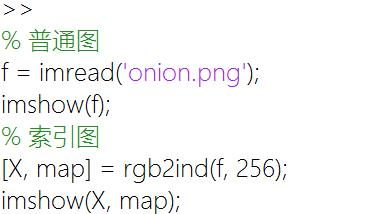输出: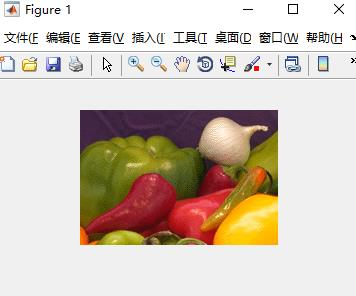图像转换

gray2ind(iamge, n) 将传入的灰度图转化索引图, n代表要分为几个索引颜色

f = imread('coins.png');
[X, map] = gray2ind(f, 5); % X为索引坐标 map为颜色索引表
imshow(X, map);

输入: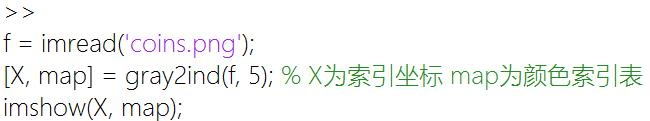输出: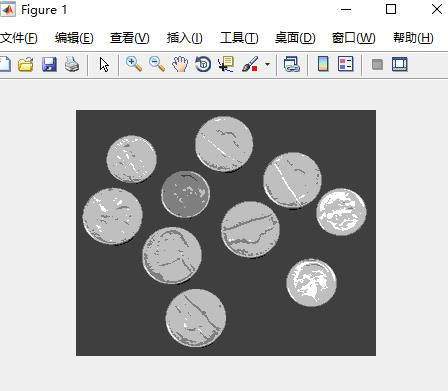rgb2gray(image) 将rgb转为gray(灰度图)

f = imread('onion.png');
g = rgb2gray(f);
imshow(g)

输入: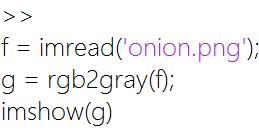输出: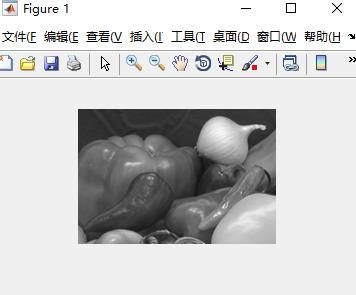im2bw(image, level) 将图转换为二值图，level为阈值(区间为[0, 1])

低于level 都为黑色（0）， 否则为白色（1）

f = imread('coins.png');
g = im2bw(f, 80/ 256);
imshow(g);

输入: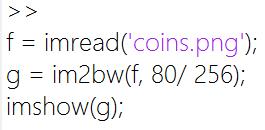输出: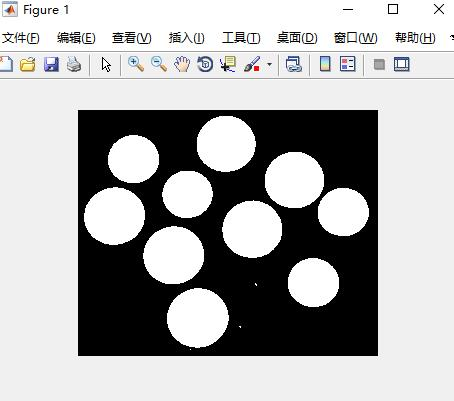ind2gray(X, map) 从索引图到灰度图

ind2rgb(X, map) 从索引图到RGB图

**mat2gray() 将矩阵转化为灰度图

f = ones(100, 100); % 创建一个100*100的矩阵
for i=1:100
for j=1:100
f(i, j) = i;
end
end
subplot(1, 2, 1), imshow(f), title('直接显示');
g = mat2gray(f);
subplot(1, 2, 2), imshow(g), title('转换后显示');

输入: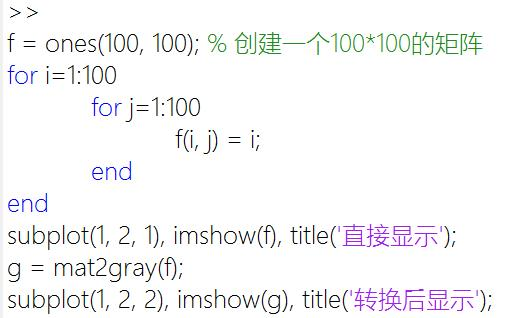输出: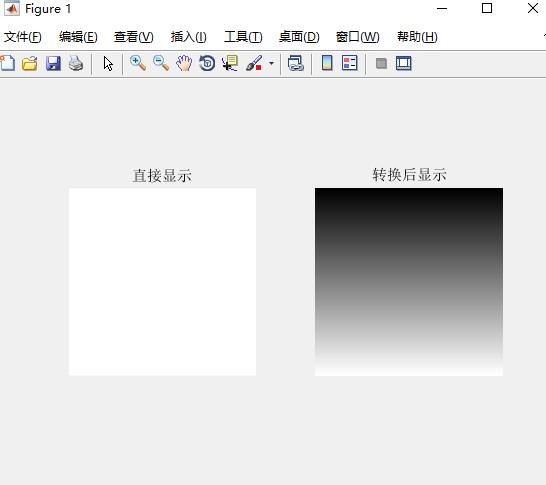图像处理

直方图

imhist(image, num) 归一化直方图

image 表示要处理的图
num表示将灰度级分为平等的几份
返回的则是灰度范围在每份灰度级中的像素个数

f = imread('onion.png');
g = rgb2gray(f);
h = imhist(g, 25);
subplot(1, 2, 1), imshow(g);
subplot(1, 2, 2), bar(h);

输入: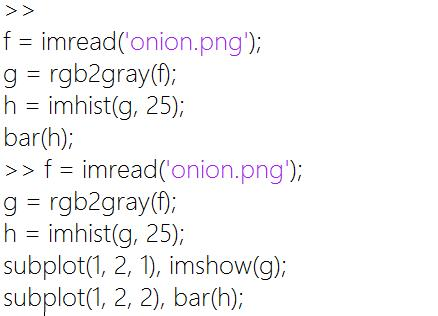输出: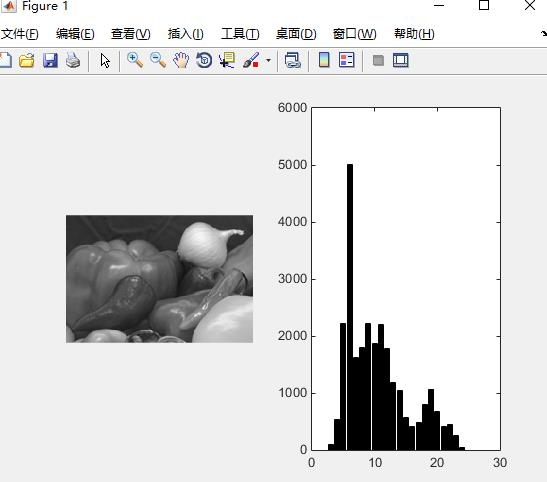bar（horz， z, width） 绘制条形图

horz 表示 水平增量，需要与z的行数相同。
z 表示要显示的条形图的数据
width 表示条形图的宽度

f = imread('onion.png');
g = rgb2gray(f);
h = imhist(g, 25) / numel(g);
subplot(2, 1, 1), imshow(g);
subplot(2, 1, 2), bar(linspace(0, 255, 25), h, 1);

输入: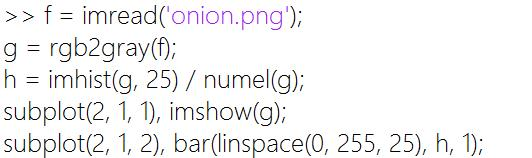输出: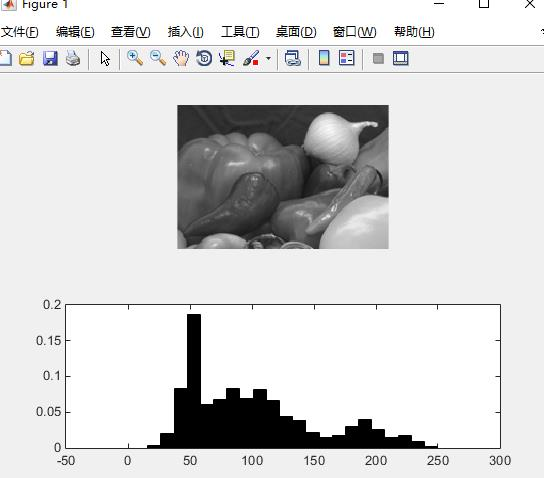stem(z) 生成一个杆状图（也可以省略h）

f = imread('onion.png');
g = rgb2gray(f);
h = imhist(g, 25) / numel(g);
subplot(1, 2, 1), stem(h);
subplot(1, 2, 2), stem(linspace(0, 1, 25), h);

输入: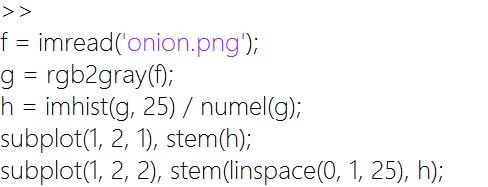输出: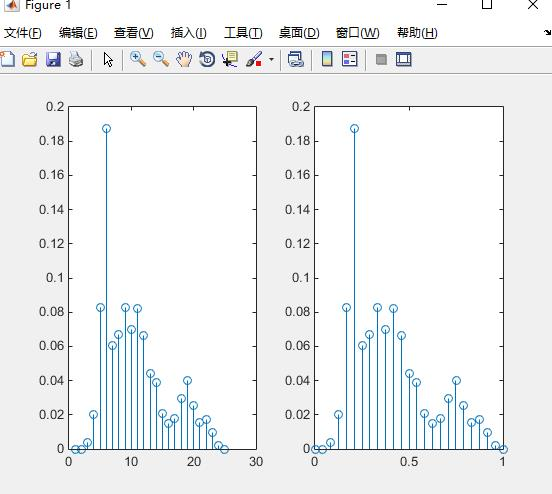stem 还有一个参数，表示杆状图以什么形式显示

stem(h, 'k:p')

具体如下
‘颜色说明符+线型说明符+标记点说明符’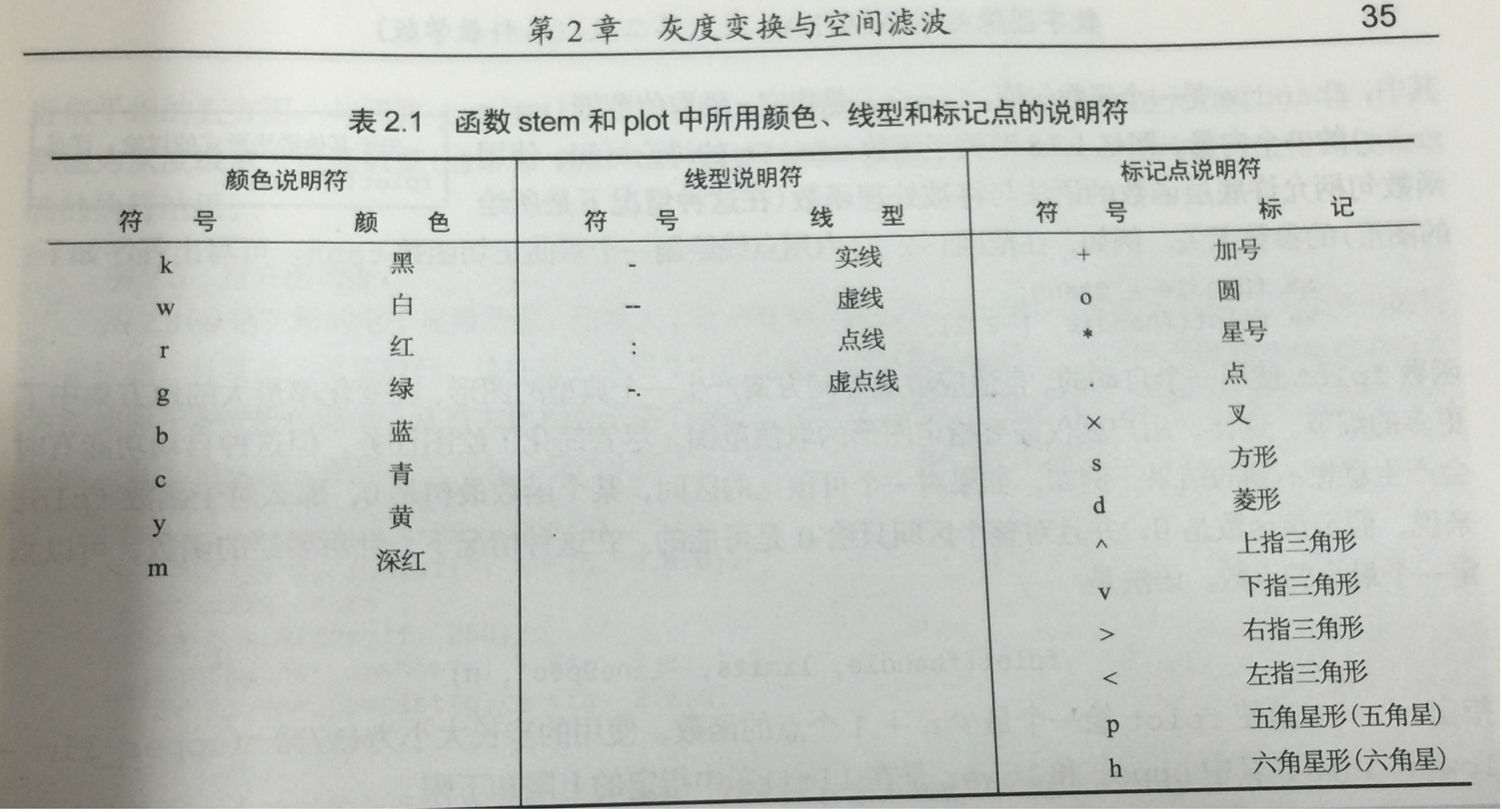plot 生成一个折线图，用法跟stem一样

f = imread('onion.png');
g = rgb2gray(f);
h = imhist(g, 25) / numel(g);
subplot(1, 2, 1), plot (h);
subplot(1, 2, 2), plot (linspace(0, 1, 25), h);

axis([xMin xMax yMin yMax]) 设置上面最近显示的图表的x轴和y轴的范围

f = imread('onion.png');
g = rgb2gray(f);
h = imhist(g, 25);
z = linspace(0, 255, 25);
bar(z, h)
axis([0 255 0 10000])

输入: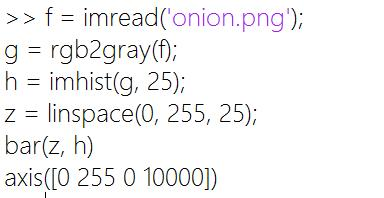输出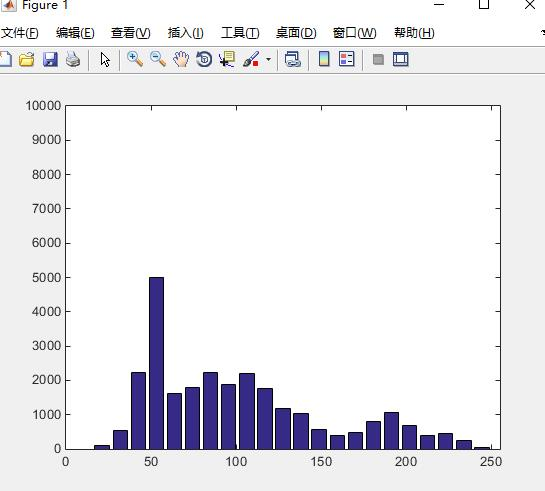接着下面可以跟着二行
set(gca, ‘xtick’, 0:50:255) % 表示将x轴的以传入的数组表示
set(gca, ‘ytick’, 0:2000:10000) % % 表示将y轴的以传入的数组表示
* 输入: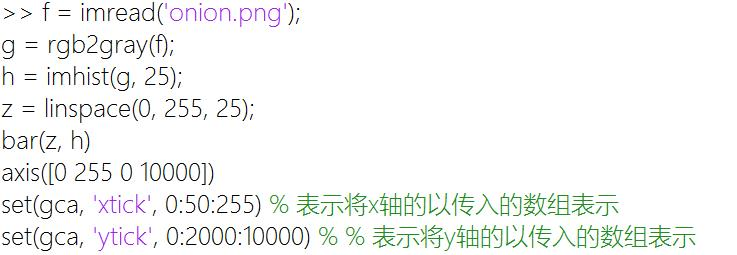* 输出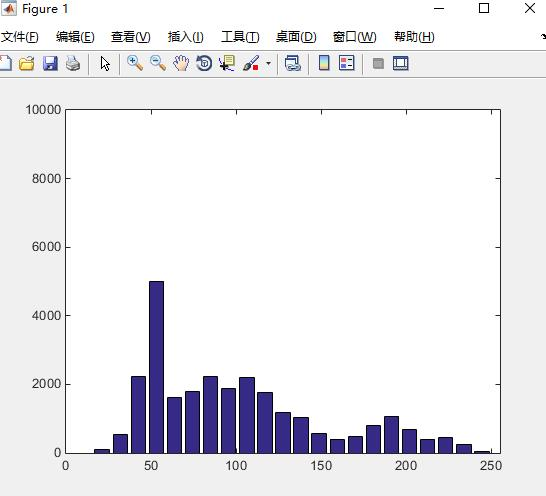axis tight 自适应图标

f = imread('onion.png');
g = rgb2gray(f);
h = imhist(g, 25);
z = linspace(0, 255, 25);
bar(z, h)
axis tight

输入: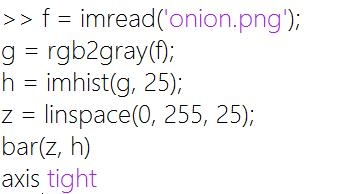输出: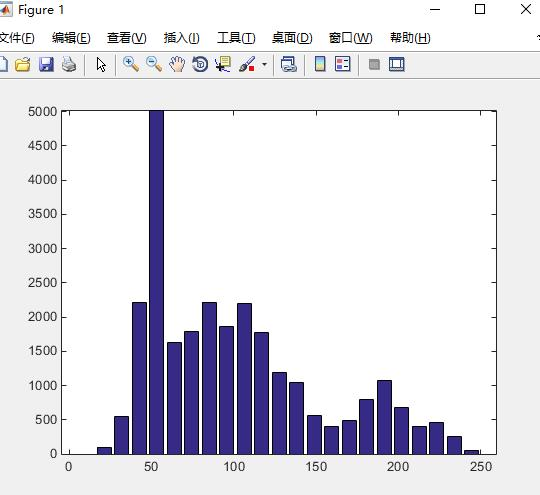xlabel ylabel 给x和y轴添加标记

xlabel('灰度级')
ylabel('次数')

text(x, y, text) 在x轴的值为x，y轴的值为y处添加text文件

text(50, 2000, '50,2000')

title(title) 给图片添加标题

title('我是可爱的标题')

输入: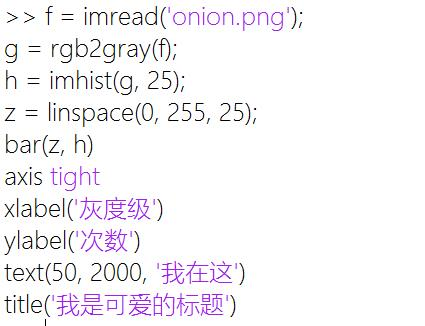输出: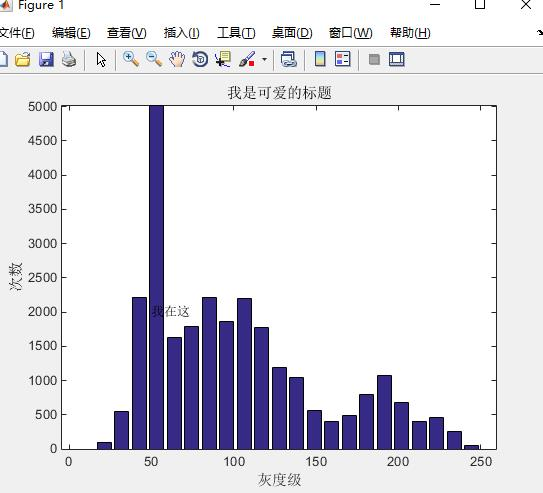hold on, hold off 开启、关闭保持当前图标状态

bar(z, h)
hold on
stem(z, h)
hold off
bar(z, h)

ylim xlim 设置y轴x轴的范围（默认就是auto状态）

ylim([0 60000])
xlim([0 255])

灰度级

imadjust(image, [low_in high_in], [low_out high_out], gamma) 用于将图像进行灰度级转换

f = imread('onion.png');
g = imadjust(f, [], [1, 0]);
imshow(g)

imcomplement(image) 获得图片的负片, 可以实现CMY模型与RGB互换

rgb = imcomplement(cmy);
cmy = imcomplement(rgb);

上面二个结果一样
* 输入: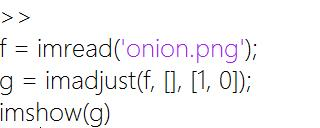* 输出: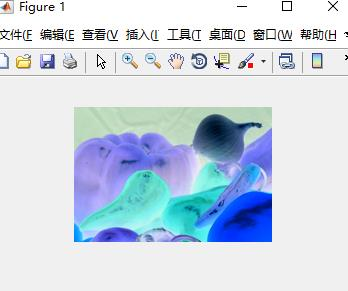stretchlim(image, tol) 用于实现对比度拉伸

f = imread('onion.png');
f = rgb2gray(f);
Low_High = stretchlim(f, [0.15 0.99]);
subplot(2,2,1), imshow(f), title('原图');
subplot(2,2,3), bar(imhist(f) / numel(f)), axis tight,title('原图 灰度图');
subplot(2,2,2), imshow(g), title('拉伸后');
subplot(2,2,4), bar(imhist(g) / numel(g)), axis tight, title('拉伸后 灰度图');

输入: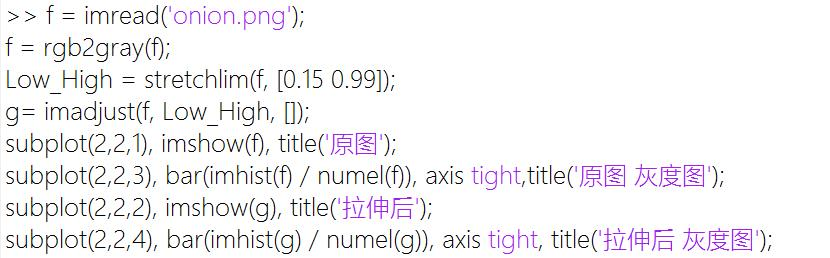输出: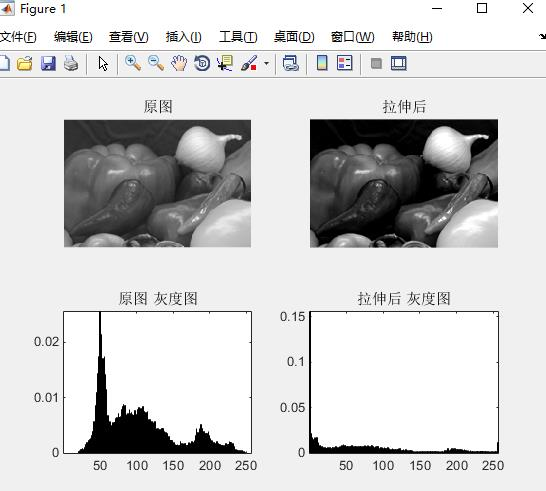graythresh(image) 获得图片最优的阈值。

f = imread('rice.png');
g = im2bw(f, graythresh(f));
subplot(1, 2, 1), imshow(f), title('原图');
subplot(1, 2, 2), imshow(g), title('二值化（最优阈值）');

输入: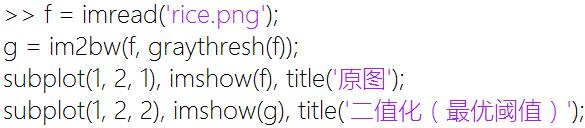输出: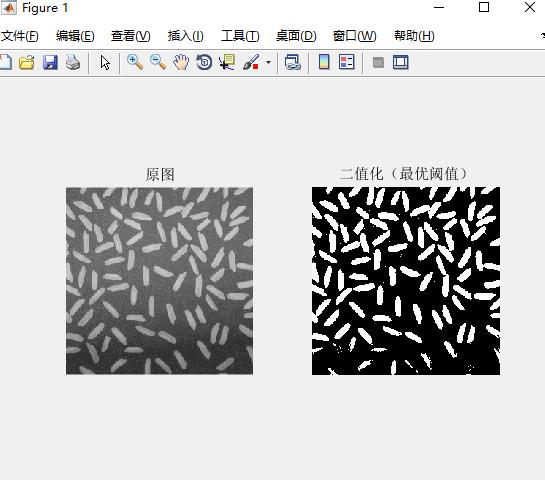histeq（image, num） 该函数可以将输入的图片按灰度级分为num份，使得每份所占的比例近似相等。

f = imread('rice.png');
g = histeq(f, 5);
t = imhist(g, 5);
subplot(1, 2, 1), bar(t); subplot(1, 2, 2), imshow(g);

输入: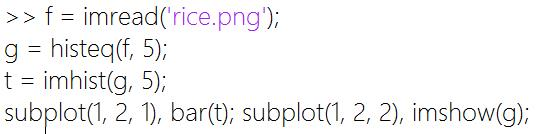输出: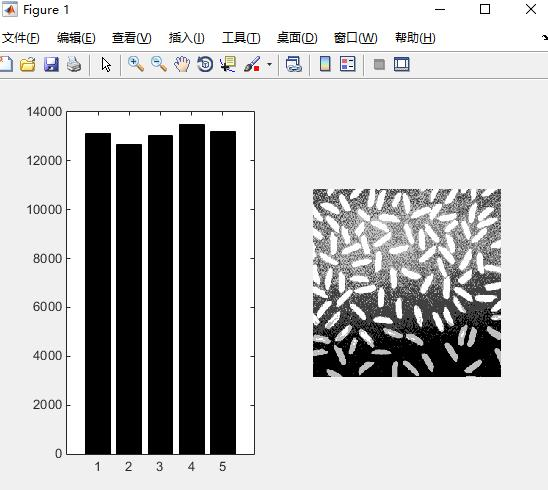f = imread('onion.png');
f = rgb2gray(f);
subplot(1, 2, 1), imshow(f), title('原图');
subplot(1, 2, 2), imshow(g), title('增强后');

输入: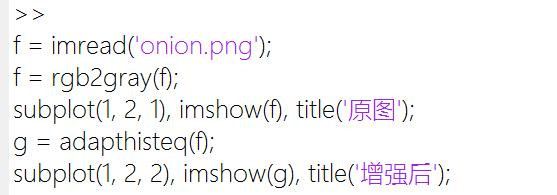输出: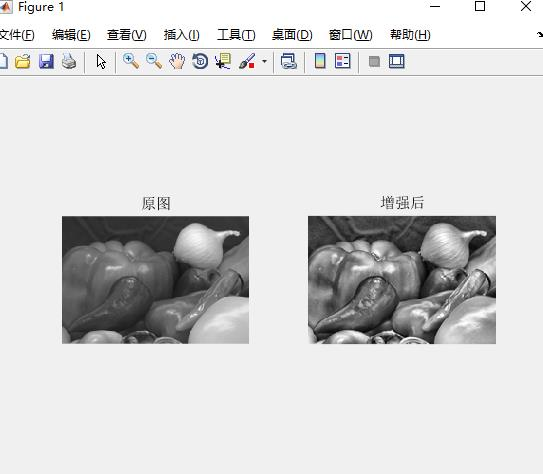imfilter(image, mod) 线性滤波

可选参数为三个，分别是滤波模式、边界选项、大小选项
滤波模式: ‘corr’ ‘conv’
边界选项: P ‘replicate’ ‘symmetric’ ‘circular’
大小选项: ‘full’ ‘same’

% 创建测试图像
f = zeros(500);
f(250:500, 1:250)=1;
f(1:250, 250:500)=1;
imwrite(f, 'temp.bmp'); % 保存测试图片
g = imfilter(f / 50 / 50, ones(50)); subplot(2, 2, 1), imshow(g);title('none');
g = imfilter(f / 50 / 50, ones(50), 'replicate'); subplot(2, 2, 2), imshow(g);title('replicate');
g = imfilter(f / 50 / 50, ones(50), 'circular'); subplot(2, 2, 3), imshow(g);title('circular');
g = imfilter(f / 50 / 50, ones(50), 'symmetric'); subplot(2, 2, 4), imshow(g);title('symmetric');

输入: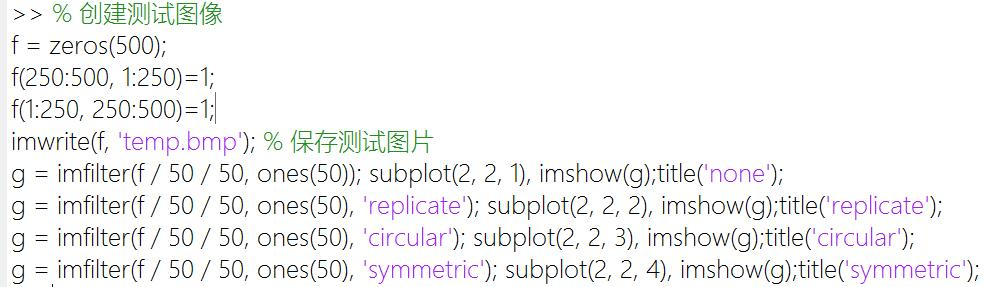输出: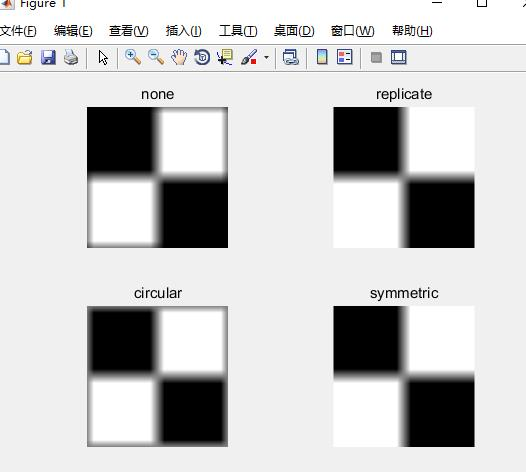colfilt(image, [m n], ‘sliding’, fun) 非线性空间滤波， 传入图片，滤波区域，和函数句柄

t = colfilt(g, [5 5], 'sliding', fun);
t = gscale(t, 'full16'); % 该函数看附录

fspecial(type, paramters) 线性空间滤波器，type值有9种情况，对应paramters也不同

type : ‘average’ ‘disk’ ‘gaussian’ ‘laplacian’ ‘log’ ‘motion’ ‘prewitt’ ‘sobel’ ‘unsharp’
paramters: [r c] r [r c], sig alpha [r c], sig len, theta null null alpha (与上面的一一对应，null为没有)

f = imread('moon.tif');
figure, imshow(f);title('none');
g = imfilter(tofloat(f), fspecial('average')); subplot(3, 3, 1), imshow(g); title('arverage 3*3');
g = imfilter(tofloat(f), fspecial('disk')); subplot(3, 3, 2), imshow(g); title('disk 5');
g = imfilter(tofloat(f), fspecial('gaussian')); subplot(3, 3, 3), imshow(g); title('gaussian 3*3 0.5');
g = imfilter(tofloat(f), fspecial('laplacian')); subplot(3, 3, 4), imshow(tofloat(f) - g); title('laplacian 0.2');
g = imfilter(tofloat(f), fspecial('log')); subplot(3, 3, 4), imshow(tofloat(f) - g); title('log 5*5 0.5');
g = imfilter(tofloat(f), fspecial('motion')); subplot(3, 3, 5), imshow(g); title('motion 9 0');
g = imfilter(tofloat(f), fspecial('prewitt')); subplot(3, 3, 6), imshow(tofloat(f) - g); title('prewitt');
g = imfilter(tofloat(f), fspecial('sobel')); subplot(3, 3, 7), imshow(tofloat(f) - g); title('sobel');
g = imfilter(tofloat(f), fspecial('unsharp')); subplot(3, 3, 8), imshow(g); title('unsharp 0.2');

参数的具体定义直接输入 doc fspecial 即可
* 输入: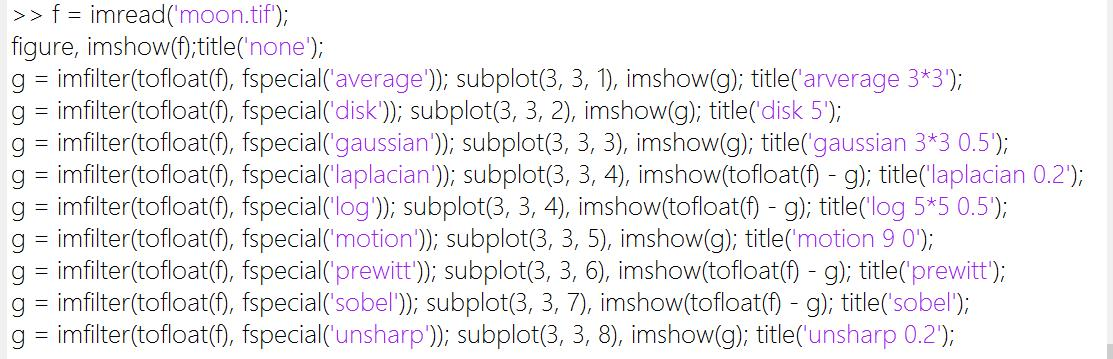* 输出: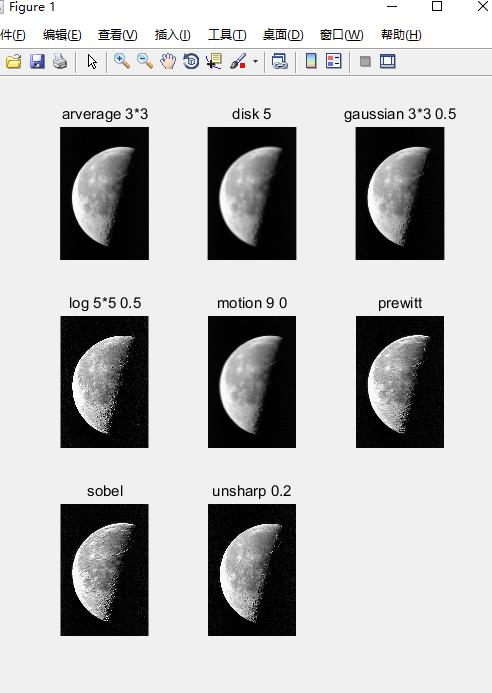f = imread('onion.png');
f = rgb2gray(f);
g = imnoise(f, 'salt & pepper', 0.2);
t = medfilt2(g); subplot(3, 3, 1), imshow(t);title('none 3*3');
t = medfilt2(g, 'zero'); subplot(3, 3, 2), imshow(t);title('zero 3*3');
t = medfilt2(g, 'symmetric'); subplot(3, 3, 3), imshow(t);title('symmetric 3*3');
t = medfilt2(g, 'indexed'); subplot(3, 3, 4), imshow(t);title('indexed 3*3');
t = medfilt2(g, [4 4]); subplot(3, 3, 5), imshow(t);title('none 4*4');
t = medfilt2(g, [4 4], 'zero'); subplot(3, 3, 6), imshow(t);title('zero 4*4');
t = medfilt2(g, [4 4], 'symmetric'); subplot(3, 3, 7), imshow(t);title('symmetric 4*4');
t = medfilt2(g, [4 4], 'indexed'); subplot(3, 3, 8), imshow(t);title('indexed 4*4');
subplot(3, 3, 9),imshow(g);title('原图');

去燥效果明显，但是具体参数的话，还是看出来太大的差别(滤波区域的设定还是很重要地)。
* 输入: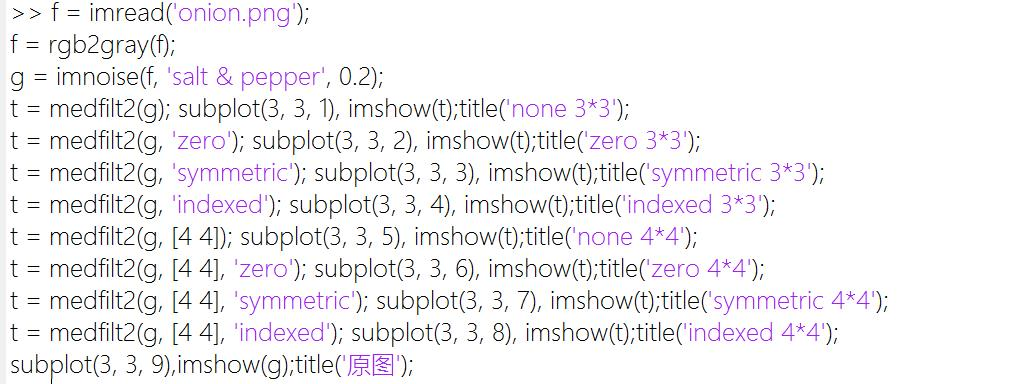* 输出: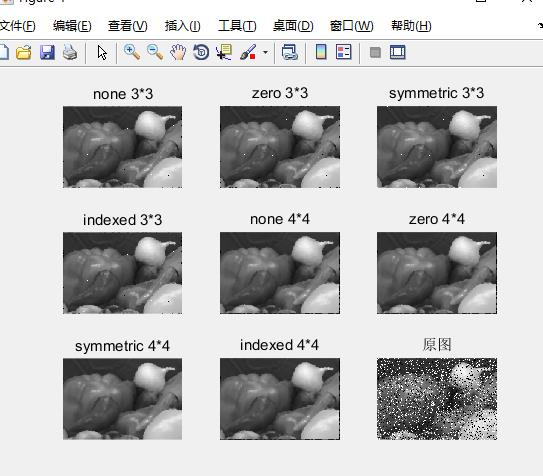输入输出

窗口

figure(h) 打开一个新窗口（将h窗口置顶，h省略时则新建一个窗口）

figure(1);
figure(2);
figure(1);
figure(2);
imshow(imread('onion.png'));

输入: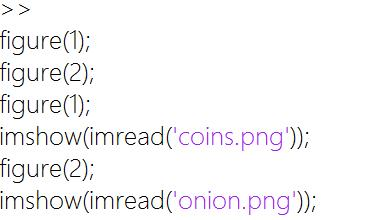输出: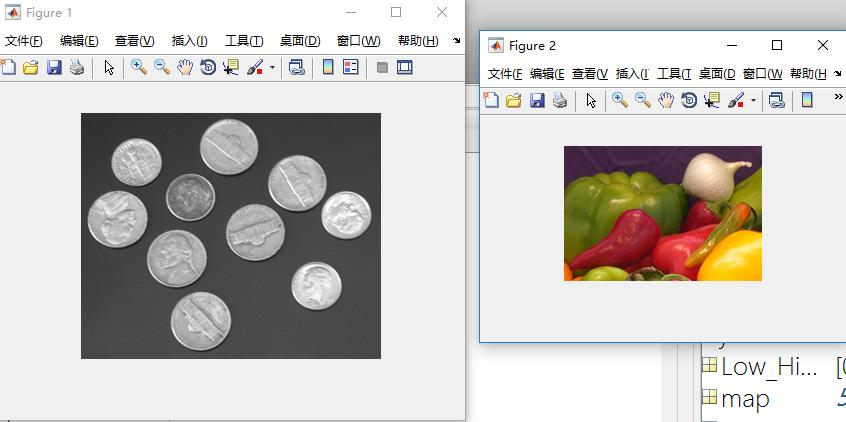subplot(h, w, id) 将最近显示的的窗口（之前没有显示的话，会新建一个窗口），分为高位h, 宽为w个单位，并指定下一个要显示的图像在这个窗口的第id个单位格；

f = imread('coins.png');
subplot(1, 3, 1), imshow(f), title('原图');
subplot(1, 3, 2), imshow(imcomplement(f)), title('负片');
subplot(1, 3, 3), imshow(im2bw(f, graythresh(f))), title('二值图');

输入: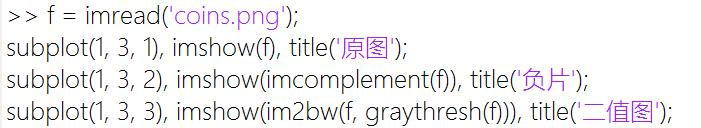输出: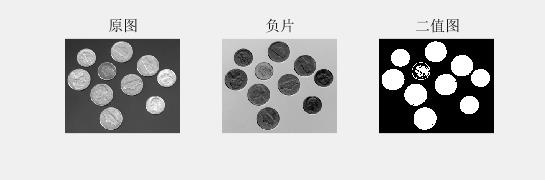控制台

disp 显示信息

disp 'Asdasd' % 输出Asdasd

input 从键盘中输入

t = input('asd', 's'); % 参数s表示将结果以字符串的形式储存

error 显示警告

error '这是错误的！' % 这里是红色警告字

附录

预设图片函数

gscale.m

function g = gscale(f, varargin)
if isempty(varargin)
method = 'full8';
else
method = varargin{1};
end

if strcmp(class(f), 'double') && (max(f(:)) > 1 || min(f(:)) < 0)
f = mat2gray(f);
end

switch method
case 'full8'
g = im2uint8(mat2gray(double(f)));
case 'full16'
g = im2uint16(mat2gray(double(f)));
case 'minmax'
low = varargin{2};
high = varargin{3};
if low > 1 || low < 0 || high > 1 || high < 0
error('low | high must be [0, 1]');
end
if strcmp(class(f), 'double')
low_in = max(f(:));
high_in = max(f(:));
elseif strcmp(class(f), 'uint8')
low_in = double(min(f(:))) ./ 255;
high_in = double(max(f(:))) ./ 255;
elseif strcmp(class(f), 'uint16')
low_in = double(min(f(:))) ./ 65535;
high_in = double(max(f(:))) ./ 65535;
end
g = imadjust(f, [low_in high_in], [low high]);

otherwise
error('Unknow method');
end

tofloat.m

function [out, revertclass] = tofloat(in)
identity = @(x) x;
tosingle = @im2single;
table = {'uint8', tosingle, @im2uint8
'uint16', tosingle, @im2uint16
'uint32', tosingle, @im2int32
'logical', tosingle, @logical
'double', identity, identity
'single', identity, identity};

classIndex = find(strcmp(class(in), table(:, 1)));
if isempty(classIndex)
error('Unsupported input image class');
end

out = table{classIndex, 2}(in);

revertclass = table{classIndex, 3};
展开全文• （一）快捷键 clc ：清空命令窗口 clear：清空工作区的所有变量
（一）快捷键
clc ：清空命令窗口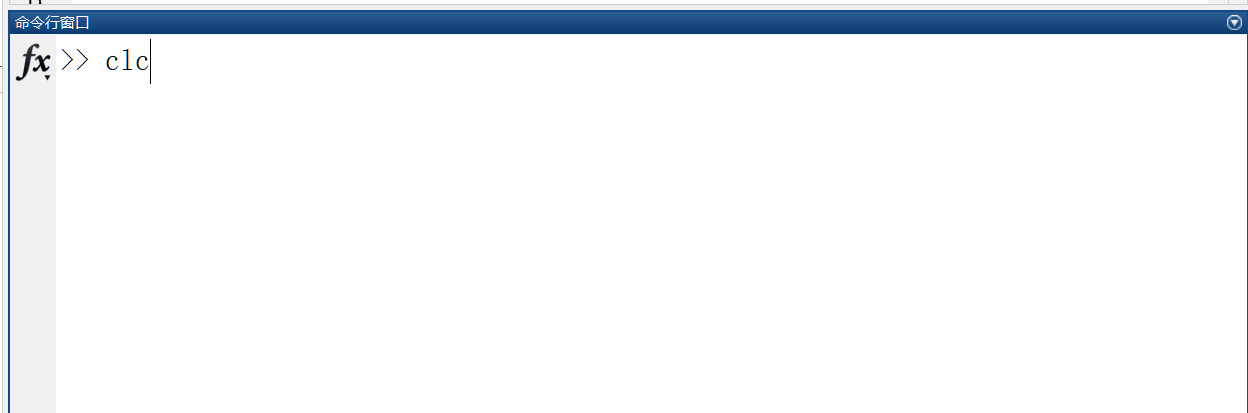clear：清空工作区的所有变量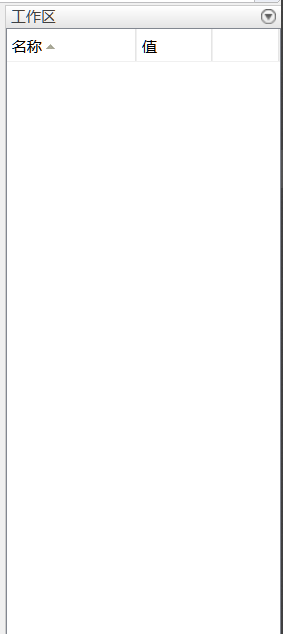展开全文• matlab学习笔记_05_数据可视化1.矩阵及其基本运算1.1矩阵的表示1.2矩阵的运算1.3矩阵分解1.4线性方程组求解1.5特征值与二次型1.6稀疏矩阵技术 1.矩阵及其基本运算 1.1矩阵的表示 sym函数–创建符号矩阵(符号矩阵的...
• matlab学习笔记 while…end 循环和fo…end 循环的区别在于：while…end 循环结构中的循环体指令组被执行的次数是不确定的，而for…end循环中的循环体被执行的次数是确定的。 for…end比while…end的性能相对...
• 一、斐波那契法 1.斐波那契法与黄金分割法不同的是，黄金是单向缩小区间的算法，斐波那契是双向收缩。 斐波那契数列指的是 0, 1, 1, 2, 3, 5, 8, 13, 21, 34, 55, 89, 144, 233，377，610，987，1597，2584，4181，...算法
• （一）简单操作 一、命令行操作 简单的说就跟C语言（更简单来说，就跟手写的一样）。 二、变量、数值与表达式 1、变量 （1）命名规则： ①变量名必须以字母开头； ②变量名可以由字母、数字和下画线混合组成，但变量...
• ## matlab学习笔记（基础）

万次阅读 多人点赞 2017-04-08 11:00:28
开头第一段怎么讲，学matlab的当前目标是看懂别人的matlab代码，所以先跳过前面一堆命令行，看看matlab代码是个什么。脚本文件首先，一个用文本形式保存的matlab代码有两种形式：matlab脚本和matlab函数，他们的扩展...
• MATLAB学习笔记（四）一、MATLAB特殊矩阵1.1 通用特殊矩阵1.2 用于专门学科的特殊矩阵1.2.1 魔方阵1.2.2 范德蒙德矩阵1.2.3 希尔伯特矩阵1.2.4 伴随矩阵1.2.5 帕斯卡矩阵二、MATLAB矩阵变换2.1 对角阵2.2 三角阵2.3 ...
• 学习书籍:《MATLAB图像处理实例详解》 掌握的知识点： rgb2gray()函数 imshow()函数 title()标题显示函数 xlabel()坐标显示函数 函数说明 rgb2gray()函数:将彩色图像转化为灰度图像（黑白图像）； imshow()...
• 3.18多维数组 3.18.1 多维数组的创建： 1.通过直接赋值来取得 例： 2.通过矩阵函数来进行生成 例： cat：矩阵连接函数 ...MATLAB的大多数内装函数支持多维数组的运算，如sum，mean，prod等 例： ...多维数组
• 本章学习内容主要有以下几个方面：- 符号变量的创建与运算 - 数学表达式的分解与化简 - 符号表达式求解 - 方程式求解 - 表达式的符号微分运算 - 表达式的积分运算一.符号代数此处掌握表达式和方程的建立和...
• MATLAB学习笔记（二）一、MATLAB变量及其操作1.1 内存变量文件的管理二、MATLAB矩阵的表示2.1 矩阵的建立2.2 创建行向量2.2.1 冒号表达式2.2.2 利用linspace()函数2.3 结构矩阵和单元矩阵2.3.1 结构矩阵2.3.2 单元...
• Curve Fitting Tool matlab中自带的曲线拟合工具，输入命令cftool调出工具界面；
• MATLAB学习笔记（八）一、二维曲线1.1 plot函数1.2 fplot函数二、绘制图形的辅助操作2.1 图形标注2.2 坐标控制2.3 图形保持2.4 图形窗口分割三、其他形式的二维图形3.1 其他坐标系下的二位曲线图3.1.1 对数坐标图...
• MATLAB学习笔记（七）一、MATLAB程序流程控制1.1 文件的建立1.2 顺序结构1.3 选择结构1.3.1 单分支if语句1.3.2 双分支if语句1.3.3 多分支if语句1.3.4 switch语句1.4 循环结构1.4.1 for语句1.4.2 while语句1.5 函数...
• MATLAB学习笔记（九）一、三维曲线1.1 plot3函数1.2 fplot3函数二、三维曲面2.1 平面网格数据的生成2.2 绘制三维曲面的函数2.3 绘制标准三维曲面三、图形修饰处理3.1 视点处理3.2 色彩处理3.3 剪裁处理四、交互式...
• MATLAB学习笔记（五）一、矩阵求值1.1 矩阵的行列式值1.2 矩阵的秩1.3 矩阵的迹1.4 矩阵的范数1.4.1 向量的范数1.4.2 矩阵的范数1.5 矩阵的条件数 一、矩阵求值 1.1 矩阵的行列式值 1.2 矩阵的秩 1.3 矩阵的迹 ...
• matlab的数据类型 一. 3.14 .matlab数据类型概述 matlab建立了不同的数据类型来满足不同用户的需求，将其进行分类，树状图大致如下： 字符型数据由单引号括起来，储存形式为ASCII码。字符型数组通常用于程序的操作...
• 用普通坐标变换的方法进行旋转图像时，由于取整后的坐标不能与原来一一对应，会出现空像素即黑点，例如I=imread('cameraman.tif'); x=pi/4; [m,n]=size(I); J=zeros(m,n); for n1=1:m for n2=1:n ...图像处理
• 一起来学matlab-matlab学习笔记11 11_2 高维数组处理和运算 squeeze, ind2sub, sub2ind 觉得有用的话,欢迎一起讨论相互学习~Follow Me squeeze 删除单维度–B=squeeze(A) B = squeeze(A)返回一个与A元素相同，但...squeeze
• 这篇笔记主要与编写MATLAB脚本有关。 MATLAB允许写两种程序文件：脚本和函数。脚本用来执行一系列命令，但是不接受输入和输出，只是为了方便执行某些特定的经常要重复用的命令而已。函数接受输入和输出，内部变量是...
• 向量、数组（这两个词在matlab中可认为同义）：1*n的矩阵 矩阵：矩阵 声明方法： *分号可以表示分行，单引号可以表示矩阵的转置 2、"ones" 命令可以生成一个全部元素为 1 的 n*n 矩阵 类似命令还有 zeros...
• 分别采用roberts、sobel、prewitt、canny、log算子来检测图像的边缘并比较 I=imread('rice.png'); B1=edge(I,'roberts'); B2=edge(I,'sobel'); B3=edge(I,'prewitt'); B4=edge(I,'canny');...subplot(231),imshow(I),...
• matlab 读取图片并转化图片格式 a imread ('路径/图片.格式')； imshow（a）； imwrite（a，图片.format）；  matlab的两个函数nargin 和 nargout；分别返回函数输入的参数和函数...图片...